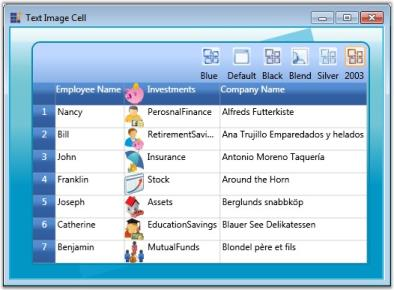# Cell Types in WPF GridControl

Essential Grid allows the inclusion of some special controls in the grid cells. This greatly improves the usability and appearance of the grid control. This attribute of a grid cell is referred to as its Cell Type.

This section lists out various cell controls that can be placed inside the grid cells. Essential Grid currently supports 20+ cell types. The cell types are broadly classified into two categories:

• Built-in cell types—Refer Built-in Cell Types section to know the procedure to add those cell types that are in-built into the grid.
• Custom cell types—Refer Custom Cell Types section to know the procedure to add add custom-derived cell types to a grid.

You can also insert images into the grid cells. Refer Inserting Images Into Grid Cells section to know the procedure to add images to a grid cell.

## Built-in Cell Types

Following is the list of built-in cell types:

### Basic Cell Types

This section elaborates you on how to employ basic controls like Check Box, Radio Button and more in a grid cell. The list of cell types and their usages are described below. The table also lists the format string for the individual cell types.

Cell Type Cell Type String Usage
Static “Static” Cannot be edited
Check Box “CheckBox” Used for toggling options
Button “Button” Provides Click event, which can be triggered to perform required action
Image “ImageCell” Used to display pictures

To set up desired cell type, the Style.CellType property must be assigned with the corresponding format string. For instance, if you want to display a Check box control in the cell (2, 2), then you have to use the code below.

Displaying a Check Box Control in a cell

``````GridStyleInfo style = gridControl1.Model[2, 2];
style.CellType = "CheckBox";``````

Likewise, you can also add other controls from the table above. A sample output is displayed below.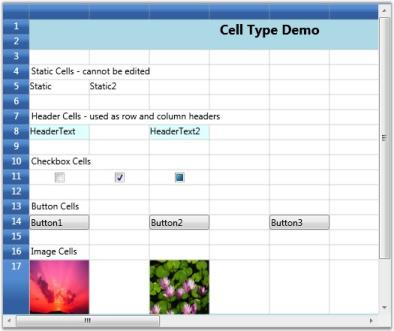Basic Cell Types

A check box is created in the grid.

NOTE

For complete code, please refer to the following browser sample: …\My Documents\Syncfusion\EssentialStudio<Version Number>\WPF\Grid.WPF\Samples\3.5\WindowsSamples\Cell Types\Basic Cell Type Demo

### Combo Box Cells

A combo box is a component with a drop-down arrow that users click to display an associated list of choices. The user displays the list by clicking or dragging the drop-down arrow.

This cell type allows you to choose the cell value from a drop-down list. You can customize this list in many ways by setting the appropriate GridStyleInfo property. Some interesting options are Autocomplete, associate a string collection, associate LINQ source etc. You can also use this drop-down like a foreign key– for example, displaying one column in the drop-down while saving the cell value from another column in the data source.

NOTE

A foreign key is a field in a relational table that matches the primary key column of another table. The foreign key can be used to cross-reference tables.

The table below lists various properties that can affect combo box cells.

GridStyleInfo Property Description
CellType Set to “ComboBox” for a Combo box control
ChoiceList String collection for the drop-down
DropDownStyle Determines the drop-down cell behavior.Editable Autocomplete Exclusive
ItemsSource Specifies the binding source for the Combo box.
Display Member String that names the public property from the data source object to be displayed in the cell.
Value Member String that names the public property from the data source object to be used as the value for this cell.

Before we proceed further the following note provides more information on the drop-down styles:

NOTE

• Editable-Editable combo boxes combine an editable text field and provide users the additional option of typing an item that might or might not be on the list. The item to be typed in the text field need not be case-sensitive. * _Autocomplete-Autocomplete combo boxes predict a word or phrase that the user wants to type in the associated text box without the user actually typing it completely. * Exclusive-This is a non-editable combo box where user is allowed to select only the options available from the drop-down list.

Combo-boxes can be added to the Grid in two different ways as follows:

1. Using ChoiceList
2. Using ItemsSource

#### Using ChoiceList

Let us see how to build different kinds of combo boxes using ChoiceList. This allows you to customize the options to be displayed in a drop-down.

Setting Up an Editable Combo Box

``````StringCollection list = new StringCollection();

//Editable Combo
var combo1 = this.grid.Model[1, 2];
combo1.CellType = "ComboBox";
combo1.ChoiceList = list;
combo1.DropDownStyle = GridDropDownStyle.Editable;``````

#### Output

The following output is generated using the code above.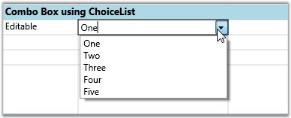Editable Combo Box using ChoiceList

An Editable Combo Box in a Grid is created

Setting Up Autocomplete Combo Box

``````//Autocomplete combo
var combo2 = this.grid.Model[2, 2];
combo2.CellType = "ComboBox";
combo2.ChoiceList = list;
combo2.DropDownStyle = GridDropDownStyle.AutoComplete;``````

#### Output

The following output is generated using the code above.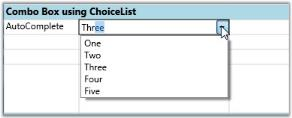Autocomplete Combo box using ChoiceList

An Autocomplete Combo Box in a Grid is created

Setting Up Exclusive Combo Box

``````//Exclusive Combo
var combo3 = this.grid.Model[3, 2];
combo3.CellType = "ComboBox";
combo3.ChoiceList = list;
combo3.DropDownStyle = GridDropDownStyle.Exclusive;``````

#### Output

The following output is generated using the code above.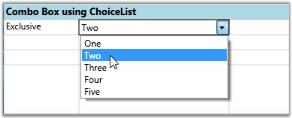Exclusive Combo box using ChoiceList

An Exclusive Combo Box in a Grid is created.

#### Using ItemsSource

The combo boxes created using ItemsSource class ensure that the options available in the drop-down list are populated from the data source the combo box is bound to. The user cannot customize the list unlike combo boxes created using ChoiceList class. The combo boxes in the following examples are bound to Northwind Employee table. The values of the FirstName column form the ItemsSource. The FirstName column is used as the display member of the combo box whose value member is EmployeeID.

Setting up editable combo box

``````//Editable Combo bound to the “FirstName” column of Northwind Employee Table.
var combo1 = this.grid.Model[4, 2];
combo1.CellType = "ComboBox";
combo1.ItemsSource = northWind.Employees.Select(emp => emp.FirstName).ToList();
combo1.DropDownStyle = GridDropDownStyle.Editable;``````

#### Output

The following output is generated using the code above.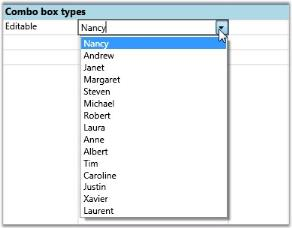Editable Combo box using ItemsSource

An editable Combo Box in a Grid is created.

Setting Up an Autocomplete Combo Box

``````//Autocomplete Combo bound to the “FirstName” column of Northwind Employee Table.
var combo2 = this.grid.Model[5, 2];
combo2.CellType = "ComboBox";
combo2.ItemsSource = northWind.Employees.Select(emp => emp.FirstName).ToList();
combo2.DropDownStyle = GridDropDownStyle.AutoComplete;
combo2.DisplayMember = "FirstName";
combo2.ValueMember = "EmployeeID";``````

#### Output

The following output is generated using the code above.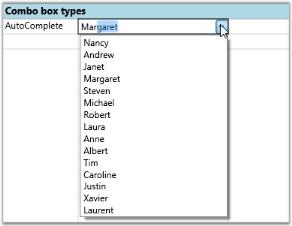Autocomplete Combo box using ItemsSource

An Autocomplete Combo Box in a Grid is created

Setting Up an Exclusive Combo Box

``````//Exclusive Combo bound to the “FirstName” column of Northwind Employee Table.
var combo3 = this.grid.Model[6, 2];
combo3.CellType = "ComboBox";
combo3.ItemsSource = northWind.Employees.Select(emp => emp.FirstName).ToList();
combo3.DropDownStyle = GridDropDownStyle.Exclusive;``````

#### Output

The following output is generated using the code above.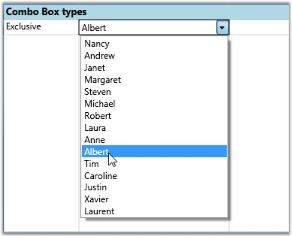Exclusive Combo box using ItemsSource

An Exclusive Combo Box in a Grid is created.

NOTE

For complete code, please refer to the following browser sample. …\My Documents\Syncfusion\EssentialStudio<Version Number>\WPF\Grid.WPF\Samples\3.5\WindowsSamples\Cell Types\Combo Box Cell Demo

This cell type serves the same purpose as combo box control. The difference is that it will associate a multicolumn drop-down to the owner cell. The other common features like DropDownStyle, ItemsSource, DisplayMember and ValueMember are applicable to this cell too.

The code snippets below allow the user to construct different List Control Cells and their output. To set up drop-down List cell, set its CellType to “DropDownList”.

Creating Editable Drop-down List Bound to LINQ Source with ‘FirstName’ as its Display Member.

``````var dropdown1 = this.grid.Model[7, 2];
dropdown1.CellType = "DropDownList";
dropdown1.ItemsSource = northWind.Employees.Select(emp =>
new
{
EmployeeID = emp.EmployeeID,
FirstName = emp.FirstName,
LastName = emp.LastName,
Phone = emp.HomePhone
}).ToList();
dropdown1.DisplayMember = "FirstName";
dropdown1.DropDownStyle = GridDropDownStyle.Editable;``````

#### Output

The following output is generated using the code above.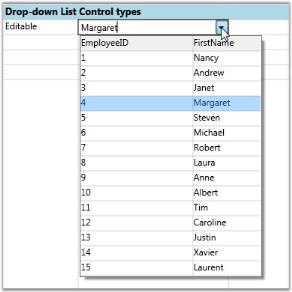Editable Drop-down List Control

An Editable drop-down list is created.

Autocomplete Drop-down List Bound to LINQ source with ‘FirstName’ as its Display Member and ‘EmployeeID’ as its ValueMember.

``````var dropdown2 = this.grid.Model[8, 2];
dropdown2.CellType = "DropDownList";
dropdown2.ItemsSource = northWind.Employees.Select(emp =>
new
{
EmployeeID = emp.EmployeeID,
FirstName = emp.FirstName,
LastName = emp.LastName,
Phone = emp.HomePhone
}).ToList();
dropdown2.DisplayMember = "FirstName";
dropdown2.ValueMember = "EmployeeID";
dropdown2.DropDownStyle = GridDropDownStyle.AutoComplete;``````

#### Output

The following output is generated using the code above.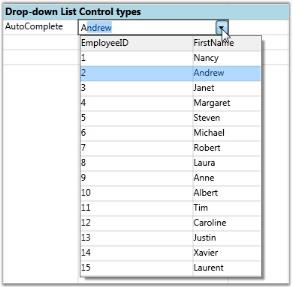Autocomplete Drop-down List Control

Exclusive Drop-down List Bound to LINQ Source with FirstName as its DisplayMember.

``````var dropdown3 = this.grid.Model[9, 2];
dropdown3.CellType = "DropDownList";
dropdown3.ItemsSource = northWind.Employees.Select(emp =>
new
{
EmployeeID = emp.EmployeeID,
FirstName = emp.FirstName,
LastName = emp.LastName,
Phone = emp.HomePhone
}).ToList();
dropdown3.DisplayMember = "FirstName";
dropdown3.DropDownStyle = GridDropDownStyle.Exclusive;``````

#### Output

The following output is generated using the code above.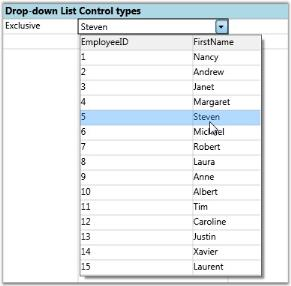Exclusive Drop-down List Control

NOTE

For complete code, please refer to the following browser sample. …\My Documents\Syncfusion\EssentialStudio<Version Number>\WPF\Grid.WPF\Samples\3.5\WindowsSamples\Cell Types\Combo Box Cell Demo

### Currency Cells

This cell type can be used to represent monetary values to achieve accuracy in the calculations. It will stripe the currency sign in the cell and attempt to parse only the number from the input. Use the GridStyleInfo properties below to customize these cells.

GridStyleInfo Property Description
Cell Type Set to “CurrencyEdit”.
CurrencyDecimalDigits Number of decimal places in currency value.
CurrencyDecimalSeparator String to use as decimal separator.
CurrencyNegativePattern Format pattern for negative currency values.
CurrencyPositivePattern Format pattern for positive currency values.
CurrencySymbol String to use as currency symbol.
CurrencyGroupSizes Number of digits in each group to the left of the decimal.

Creating a Currency Cell with a Negative Currency Value with ‘.’ as the Decimal Separator.

``````int[] sizes = { 2, 3, 4 };
grid.Model[6, 2].CellType = "CurrencyEdit";
grid.Model[6, 2].IsEditable = true;
grid.Model[6, 2].NumberFormat = new NumberFormatInfo
{
CurrencyDecimalDigits = 4,
CurrencyDecimalSeparator = ".",
CurrencyNegativePattern = 0,
CurrencyPositivePattern = 0,
CurrencySymbol = "\$"
};

grid.Model[6, 2].NumberFormat.CurrencyGroupSizes = sizes;
grid.Model[6, 2].CellValue = -4.0;``````

#### Output

The following output is generated using the code above.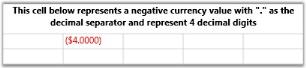Currency Cell

Currency Cell with a Negative Currency Value and a Different Negative Pattern

``````int[] sizes = { 2, 3, 4 };
grid.Model[10, 2].CellType = "CurrencyEdit";
grid.Model[10, 2].IsEditable = true;
grid.Model[10, 2].NumberFormat = new NumberFormatInfo
{
CurrencyDecimalDigits = 2,
CurrencyDecimalSeparator = ".",
CurrencyNegativePattern = 5,
CurrencyPositivePattern = 1,
CurrencySymbol = "\$"
};

grid.Model[10, 2].NumberFormat.CurrencyGroupSizes = sizes;
grid.Model[10, 2].CellValue = -14.0;``````

#### Output

The following output is generated using the code above.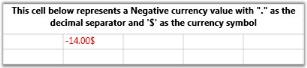Currency Cell

Currency Cell with a Positive Currency Value with ‘.’ as the Decimal Separator and ‘\$’ as Currency Symbol

``````int[] sizes = { 2, 3, 4 };
grid.Model[14, 2].CellType = "CurrencyEdit";
grid.Model[14, 2].IsEditable = true;
grid.Model[14, 2].NumberFormat = new NumberFormatInfo
{
CurrencyDecimalDigits = 4,
CurrencyDecimalSeparator = ".",
CurrencyNegativePattern = 11,
CurrencyPositivePattern = 2,
CurrencySymbol = "\$"
};

grid.Model[14, 2].NumberFormat.CurrencyGroupSizes = sizes;
grid.Model[14, 2].CellValue = 36.0;``````

#### Output

The following output is generated using the code above.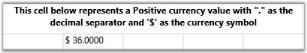Currency Cell with a Positive Value

NOTE

For complete code, please refer to the following browser sample.

…\My Documents\Syncfusion\EssentialStudio<Version Number>\WPF\Grid.WPF\Samples\3.5\WindowsSamples\Cell Types\Currency Cell Demo

### Date Time Cells

The Date Time cells incorporate DateTimeEdit controls in grid cells that will help you to interactively set a date and time value. The style properties below are applicable to this cell type.

GridStyleInfo Property Description
CellType Set to “DateTimeEdit”
DateTimePattern Sets the date-time pattern. The table below lists the available patterns with examples.
MaxDateTime, MinDateTime Sets the maximum and minimum values for a DateTime cell.
IsCalendarEnabled When true, enables the calendar popup
IsWatchEnabled When true, enables the watch popup
NoneDateText Specifies the text to be displayed when no date is set
Date and Time Pattern Example
Short Date 8/6/2009
Long Date Thursday, August 06, 2009
Long Time 7:01:33 AM
Short Time 7:01 AM
Full Date Time Thursday, August 06, 2009 7:01:33 AM
MonthDay August 06
RFC1123 Thu, 06 Aug 2009 07:01:33 GMT
Sortable Date Time 2009-08-06T07:01:33
Universal Sortable Date Time 2009-08-06 07:01:33Z
Year Month (August, 2009 is correct)August, 2009

Setting Date and Time Cells with Different Date Time Patterns.

``````grid.Model[5, 1].CellType = "DateTimeEdit";
grid.Model[5, 1].DateTimeEdit.DateTimePattern = DateTimePattern.LongTime;
grid.Model[5, 1].CellValue = DateTime.Now;

grid.Model[7, 1].CellType = "DateTimeEdit";
grid.Model[7, 1].DateTimeEdit.DateTimePattern = DateTimePattern.FullDateTime;
grid.Model[7, 1].CellValue = DateTime.Now;

grid.Model[9, 1].CellType = "DateTimeEdit";
grid.Model[9, 1].DateTimeEdit.DateTimePattern = DateTimePattern.ShortDate;
grid.Model[9, 1].CellValue = DateTime.Now;

grid.Model[11, 1].CellType = "DateTimeEdit";
grid.Model[11, 1].DateTimeEdit.DateTimePattern = DateTimePattern.YearMonth;
grid.Model[11, 1].CellValue = DateTime.Now;``````

#### Output

The following output is generated using the code above.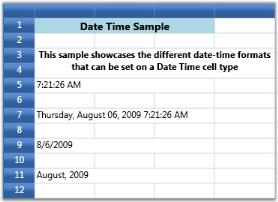DateTime Cell

NOTE

For complete code, please refer to the following browser sample. …\My Documents\Syncfusion\EssentialStudio<Version Number>\WPF\Grid.WPF\Samples\3.5\WindowsSamples\Cell Types\Date Time Cell Demo

### Double Edit Cells

Using DoubleEdit cell type will restrict the user to enter only double (value type) values into the cell. Thus it can be used to display System.Double type values. Below are the style properties that affect this cell.

GridStyleInfo Property Description
Cell Type Set to “DoubleEdit”
NumberGroupSeparator String that separates groups of digits to the left of the decimal
NumberDecimalSeparator String to use as decimal separator
NumberDecimalDigits Number of decimal places

#### Example

Setting up four Double Edit cells using different group separators and decimal digits.

``````int[] sizes = { 2, 3, 4 };
grid.Model[6, 2].CellType = "DoubleEdit";
grid.Model[6, 2].NumberFormat = new NumberFormatInfo
{
NumberGroupSeparator = ";",
NumberDecimalSeparator = ".",
NumberDecimalDigits = 4
};

grid.Model[6, 2].NumberFormat.NumberGroupSizes = sizes;
grid.Model[6, 2].CellValue = 2345.00;

grid.Model[8, 2].CellType = "DoubleEdit";
grid.Model[8, 2].NumberFormat = new NumberFormatInfo
{
NumberGroupSeparator = ",",
NumberDecimalSeparator = ".",
NumberDecimalDigits = 4
};

grid.Model[8, 2].NumberFormat.NumberGroupSizes = sizes;
grid.Model[8, 2].CellValue = 12;

grid.Model[10, 2].CellType = "DoubleEdit";
grid.Model[10, 2].NumberFormat = new NumberFormatInfo
{
NumberGroupSeparator = ",",
NumberDecimalSeparator = ".",
NumberDecimalDigits = 1
};

grid.Model[10, 2].NumberFormat.NumberGroupSizes = sizes;
grid.Model[10, 2].CellValue = 100;

grid.Model[12, 2].CellType = "DoubleEdit";
grid.Model[12, 2].NumberFormat = new NumberFormatInfo
{
NumberGroupSeparator = "@",
NumberDecimalSeparator = ".",
NumberDecimalDigits = 0
};
grid.Model[12, 2].NumberFormat.NumberGroupSizes = sizes;
grid.Model[12, 2].CellValue = 12345678.00;.00;``````

#### Output

The following output is generated using the code above.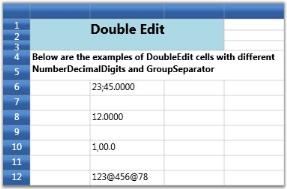Double Edit Cell

NOTE

For complete code, please refer to the following browser sample.

…\My Documents\Syncfusion\EssentialStudio<Version Number>\WPF\Grid.WPF\Samples\3.5\WindowsSamples\Cell Types\Double Edit Cell Demo

### Integer Edit Cells

IntegerEdit is a specialized cell type that restricts the data entry to integers. The table below lists the style properties specific to this cell type.

GridStyleInfo Property Description
Cell Type Set to “IntegerEdit”
NumberGroupSeparator String that separates groups of digits
NumberGroupSizes Number of digits in each group
UseNullOption By default, the value of IntegerEdit cells is "0". Set

UseNullOption

as "True" to hide or delete the default value in IntegerEdit cells.

#### Example

Setting up Three Different Integer Edit Cells.

``````int[] sizes = { 2, 3, 4 };
grid.Model[12, 2].CellType = "IntegerEdit";
grid.Model[12, 2].IsEditable = true;
grid.Model[12, 2].NumberFormat = new NumberFormatInfo { NumberGroupSeparator = ","};
grid.Model[12, 2].NumberFormat.NumberGroupSizes = sizes;
grid.Model[12, 2].CellValue = 1;

grid.Model[8, 2].CellType = "IntegerEdit";
grid.Model[8, 2].IsEditable = true;
grid.Model[8, 2].NumberFormat = new NumberFormatInfo { NumberGroupSeparator = ";"};
grid.Model[8, 2].NumberFormat.NumberGroupSizes = sizes;
grid.Model[8, 2].CellValue = 222222;

grid.Model[10, 2].CellType = "IntegerEdit";
grid.Model[10, 2].IsEditable = true;
grid.Model[10, 2].NumberFormat = new NumberFormatInfo { NumberGroupSeparator = "@"};
grid.Model[10, 2].NumberFormat.NumberGroupSizes = sizes;
grid.Model[10, 2].CellValue = 1000;

grid.Model.ColStyles.CellType = "IntegerEdit";
grid.Model.ColStyles.IntegerEdit.UseNullOption = true;``````

#### Output

The following output is generated using the code above.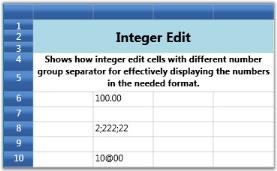Integer Edit

NOTE

For complete code, please refer to the following browser sample. …\My Documents\Syncfusion\EssentialStudio<Version Number>\WPF\Grid.WPF\Samples\3.5\WindowsSamples\Cell Types\Integer Edit Cell Demo

MaskEdit cell type allows you to create specially formatted text cells that confirm to an edit mask that you specify. The Style.MaskEdit.Mask property holds the mask string, which will control the format of the input text being entered. The Mask Edit cells are useful when the user wants to display some formatted text such as Social Security Number (SSN), telephone number etc.

#### Example

``````var maskStyleInfo = this.grid.Model[6, 2];

#### Output

The following output is generated using the code above.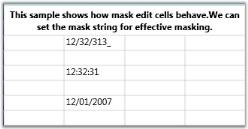NOTE

For complete code, please refer to the following browser sample. …\My Documents\Syncfusion\EssentialStudio<Version Number>\WPF\Grid.WPF\Samples\3.5\WindowsSamples\Cell Types\Mask Edit Cell Demo

### Percent Edit Cells

The PercentEdit cell type restricts the data entry to percentage values only. The following are the style properties used with this cell type.

GridStyleInfo Property Description
Cell Type Set to “PercentEdit”.
PercentEditMode Indicates the way of editing the text in percent edit cells. Possible values – PercentMode and DoubleMode
PercentSymbol String to use as the percent symbol.
PercentGroupSizes Number of digits in each group to the left of the decimal.
PercentGroupSeparator String that separates group of digits to the left of the decimal.
PercentDecimalDigits Number of digits that appear after the decimal.

#### Example

Setting up two Percent Edit cells with different group sizes and decimal digits.

The first cell operates in Percent mode of editing while the second cell follows Double mode.

Double mode displays the values in System.Double format and Percent mode adds a percent sign next to the numbers.

``````var percentStyleInfo = this.grid.Model[7, 2];
percentStyleInfo.CellType = "PercentEdit";

percentStyleInfo.NumberFormat = new NumberFormatInfo()
{
PercentSymbol = "%",
PercentGroupSizes = new int[] { 1, 2,  3 },
PercentDecimalDigits = 2,
PercentGroupSeparator = ",",
};
percentStyleInfo.PercentEditMode = PercentEditMode.PercentMode;
percentStyleInfo.CellValue = 19;
var percentStyleInfo2 = this.grid.Model[9, 2];
percentStyleInfo2.CellType = "PercentEdit";

percentStyleInfo2.NumberFormat = new NumberFormatInfo()
{
PercentSymbol = "%",
PercentGroupSizes = new int[] { 3 },
PercentDecimalDigits = 4,
PercentGroupSeparator = ",",
};
percentStyleInfo2.PercentEditMode = PercentEditMode.DoubleMode;
percentStyleInfo2.CellValue = 91;``````

#### Output

The following output is generated using the code above.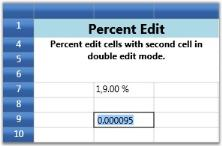Percent Edit Cell

NOTE

For complete code, please refer to the following browser sample. …\My Documents\Syncfusion\EssentialStudio<Version Number>\WPF\Grid.WPF\Samples\3.5\WindowsSamples\Cell Types\Percent Edit Cell Demo

### RichTextBox CellType

RichTextBox CellType is used to format the cells, where each character, word or a line can be given different formats. RichTextBox cell type also supports Printing, Importing and Exporting.

RichTextBox CellType can be defined in the Grid using the following code snippet:

``````//Cell type as RichText and Cell Value as FlowDocument
this.grid.Model[rowIndex, colIndex].CellType = "RichText";
this.grid.Model[rowIndex, colIndex].CellValue = _flowDocument;

//The Cell Value for RichTextBox must be in FlowDocument as shown below.

// Flow document type is supported for Rich Text Cell Type.
FlowDocument _flowDocument = new FlowDocument();
Paragraph _paragraph = new Paragraph();

Run _run1 = new Run();
_run1.Text = "This is RichText box Cell Type";
_run1.TextDecorations = TextDecorations.Underline;

Run _run2 = new Run();
_run2.Text = "Various formatting can be done in Single Cell.";
_run1.FontWeight = FontWeights.Bold;
_run2.Foreground = Brushes.Green;

Run _run3 = new Run();
_run3.Text = "Rich Text cell type also supports Images";
_run3.FontSize = 16;
_run3.FontStyle = FontStyles.Italic;

//Cell type as RichText and Cell Value as FlowDocument
this.grid.Model[rowIndex, colIndex].CellType = "RichText";
this.grid.Model[rowIndex, colIndex].CellValue = _flowDocument;``````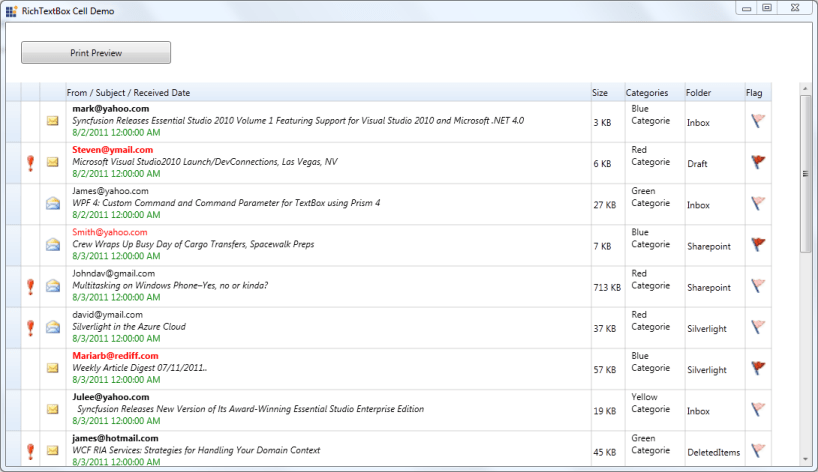RichTextBox CellType

### Up Down Edit Cells

UpDownEdit cell type makes the grid cell to host an Up and Down edit control which contains a pair of arrow buttons that increase or decrease the cell value. The style properties applicable to this cell type are provided below.

GridStyleInfo Property Description
Cell Type Set to “UpDownEdit”
NumberGroupSeparator String that separates group of digits to the left of the decimal
NumberDecimalDigits Number of digits that appear after the decimal
MaxValue Upper limit in the range of applicable values
MinValue Lower limit in the range of applicable values
Step Unit value that is to be increased or decreased when the spin buttons are clicked
FocusedBorderBrush Border brush; applied only when the cell is in focus
FocusedForeground Foreground brush; applied only when the cell is in focus
FocusedBackground Background brush; applied only when the cell is in focus

#### Example

The code below sets up two different Up and Down controls in grid cells.

``````var updownStyleInfo = this.grid.Model[6, 2];
updownStyleInfo.CellType = "UpDownEdit";
updownStyleInfo.NumberFormat = new NumberFormatInfo { NumberGroupSeparator = " ", NumberDecimalDigits = 3 };
updownStyleInfo.UpDownEdit.FocusedBackground = Brushes.Tan;
updownStyleInfo.UpDownEdit.FocusedBorderBrush = Brushes.Red;
updownStyleInfo.UpDownEdit.FocusedForeground = Brushes.Yellow;
updownStyleInfo.UpDownEdit.MaxValue = 10.00;
updownStyleInfo.UpDownEdit.MinValue = 0;
updownStyleInfo.CellValue = 10.000;

var updownStyleInfo1 = this.grid.Model[8 , 2];
updownStyleInfo1.CellType = "UpDownEdit";
updownStyleInfo1.NumberFormat = new NumberFormatInfo { NumberGroupSeparator = " ", NumberDecimalDigits = 3 };
updownStyleInfo1.UpDownEdit.FocusedBackground = Brushes.BlueViolet ;
updownStyleInfo1.UpDownEdit.FocusedBorderBrush = Brushes.Red;
updownStyleInfo1.UpDownEdit.FocusedForeground = Brushes.Bisque ;
updownStyleInfo1.UpDownEdit.MaxValue = 100.00;
updownStyleInfo1.UpDownEdit.MinValue = 0;
updownStyleInfo1.CellValue = 10.000;``````

#### Output

The following output is generated using the code above.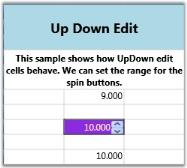Up Down Edit

NOTE

For complete code, please refer to the following browser sample. …\My Documents\Syncfusion\EssentialStudio<Version Number>\WPF\Grid.WPF\Samples\3.5\WindowsSamples\Cell Types\UpDown Cell Demo

### Nested Grid Cells

Nested grids are an important component of the basic architecture of Essential Grid. They provide for the easy display of complex user interfaces using a flat grid. They also form the underpinnings for the display of hierarchical and grouped data. You can nest grids inside a row, column or covered range. When you nest a grid inside a covered range you can specify whether the rows or columns derive their state from the parent control. You have multiple independent options for both rows and columns.

#### API definition

GridCellNestedGridModel is the class to be used as model class for this cell type. Its constructor accepts two objects of type GridNestedAxisLayout enum, where the first parameter corresponds to row and second parameter corresponds to grid column. This enum value determines whether to share the row layout or column layout or the rows and columns are independent of parent grid.

#### Nested Grid inside a Row of Parent Grid

In this case, the grid will maintain its own row heights. When you resize rows the grid will also notify the parent grid that its total height is changed. While scrolling you can scroll row by row through the nested grid. The nested grid will have no separate scrollbars. They are shared with the parent grid.

#### Example

The code below implements a nested scroll grid. The GridCellNestedScrollGridModel is the model class to be used.

``````// Add Nested Scroll Grid cell model.
GridCellNestedScrollGridModel scrollGridModel = new GridCellNestedScrollGridModel();
Model[40, 2].CellType = "ScrollGrid";

// Create scroll nested grid.
GridModel nestedGrid = new GridModel();
nestedGrid.Options.AllowSelection = GridSelectionFlags.Cell;
nestedGrid.RowHeights.DefaultLineSize = 20;
nestedGrid.RowCount = 50;
nestedGrid.ColumnWidths.DefaultLineSize = 50;
nestedGrid.ColumnCount = 12;

for (int n = 0; n < nestedGrid.RowCount; n++)
{

for (int c = 0; c < nestedGrid.ColumnCount; c++)
{
GridStyleInfo ci = new GridStyleInfo();
ci.CellType = "TextBox";
ci.CellValue = String.Format("Scroll{0}:{1}", n, c);
nestedGrid.Data[n, c] = ci.Store;
}
}
Model[40, 2].CellValue = nestedGrid;

#### Output

The following output is generated using the code above.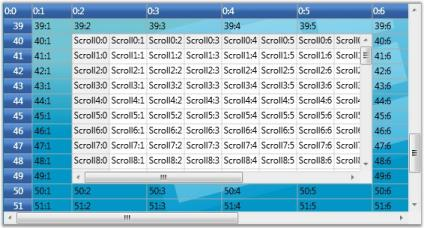Nested Grid

The same way you can nest a grid inside a complete row you can also nest a grid inside a whole column.

Nested Grid Inside a Covered Range with its Rows Tied to the Rows of the Parent Grid

In this case, the grid will have its own unique column widths but the row heights are shared with the parent grid. When scrolling through rows in the nested grid you also scroll the rows in the parent grid to keep them in sync. The nested grid will have no separate scrollbars. They are shared with the parent grid. When you resize rows they will also be resized in the parent grid and vice versa.

#### Example

The codes below show a grid whose cell contains a nested grid, which again contains a nested grid in its cell, and this second nested grid again contains a nested grid in its cell and thus forming four grids nested within one another.

To specify shared row layout, use Shared option of GridNestedAxisLayout enum in the first parameter.

``````// Add appropriate Nested Grid cell model.
GridCellNestedGridModel shareRow = new GridCellNestedGridModel (GridNestedAxisLayout.Shared, GridNestedAxisLayout.Normal);

// Setup nested grid with shared row layout
Model[100, 2].CellType = "ShareRowLayoutGrid";
Model[100, 2].BorderMargins.Top = 0;
Model[100, 2].BorderMargins.Left = 0;
Model[100, 2].BorderMargins.Right = 0;
Model[100, 2].BorderMargins.Bottom = 0;
Model[100, 2].Background = SystemColors.InactiveCaptionBrush;
GridModel nestedGridWithSharedRowsModel = GetNestedGridWithSharedRowsModel();
Model[100, 2].CellValue = nestedGridWithSharedRowsModel;
CoveredCells.Add(new CoveredCellInfo(100, 2, 100 + nestedGridWithSharedRowsModel.RowCount - 1, 5));

// Setup the top level(parent) nested grid

private GridModel GetNestedGridWithSharedRowsModel()
{
GridModel model = new GridModel();
Pen gridLinePen = new Pen(Brushes.DarkGray, 1);
gridLinePen.Freeze();
model.Options.AllowSelection = GridSelectionFlags.Cell;
model.ColumnWidths.DefaultLineSize = 50;
model.ColumnCount = 12;
model.RowHeights.FooterLineCount = 1;
model.RowCount = 601;
Color clr = Color.FromArgb(128, 0, 0, 0);
Color clr2 = Color.FromArgb(128, 128, 0, 0);
Brush footerBrush = new SolidColorBrush(clr2);
footerBrush.Freeze();

for (int n = 0; n < model.RowCount; n++)
{

for (int c = 0; c < model.ColumnCount; c++)
{
GridStyleInfo ci = new GridStyleInfo();
ci.CellType = "TextBox";
ci.CellValue = String.Format("{0}:{1}", n, c);
ci.BorderMargins.Top = gridLinePen.Thickness;
ci.BorderMargins.Left = gridLinePen.Thickness;
ci.BorderMargins.Right = gridLinePen.Thickness / 2;
ci.BorderMargins.Bottom = gridLinePen.Thickness / 2;
ci.Borders.Right = gridLinePen;
ci.Background = null;// Brushes.White;
ci.Borders.Bottom = gridLinePen;
model.Data[n, c] = ci.Store;

if (c == 0 || n == 0)
{
ci.CellType = "Static";
}

if (n == model.RowCount - 1)
{
ci.CellType = "Static";
ci.Background = footerBrush;
}
}
}
GridModel nestedGridWithSharedRowsModel = GetSecondNestedGridWithSharedRowsModel();
model[10, 2].CellType = "ShareRowLayoutGrid";
model[10, 2].BorderMargins.Top = 0;
model[10, 2].BorderMargins.Left = 0;
model[10, 2].BorderMargins.Right = 0;
model[10, 2].BorderMargins.Bottom = 0;
model[10, 2].Background = SystemColors.InactiveCaptionBrush;

// Creates a nested grid for second level.
model[10, 2].CellValue = nestedGridWithSharedRowsModel;
model.CoveredCells.Add(new CoveredCellInfo(10, 2, 10 + nestedGridWithSharedRowsModel.RowCount - 1, 7));
model.SelectedCells = GridRangeInfo.Empty;
return model;
}

// Setup the second level nested grid

private GridModel GetSecondNestedGridWithSharedRowsModel()
{
GridModel model = new GridModel();
Pen gridLinePen = new Pen(Brushes.DarkGray, 1);
gridLinePen.Freeze();
model.Options.AllowSelection = GridSelectionFlags.Cell;
model.ColumnWidths.DefaultLineSize = 40;
model.ColumnCount = 8;
model.RowHeights.FooterLineCount = 1;
model.RowCount = 121; // make sure this matched covered cell size ...
Color clr = Color.FromArgb(128, 0, 0,128);
Color clr2 = Color.FromArgb(128, 0, 128, 0);
Brush footerBrush = new SolidColorBrush(clr2);
footerBrush.Freeze();

for (int n = 0; n < model.RowCount; n++)
{

for (int c = 0; c < model.ColumnCount; c++)
{
GridStyleInfo ci = new GridStyleInfo();
ci.CellType = "TextBox";
ci.CellValue = String.Format("{0}:{1}", n, c);
ci.BorderMargins.Top = gridLinePen.Thickness;
ci.BorderMargins.Left = gridLinePen.Thickness;
ci.BorderMargins.Right = gridLinePen.Thickness / 2;
ci.BorderMargins.Bottom = gridLinePen.Thickness / 2;
ci.Borders.Right = gridLinePen;
ci.Background = null;// Brushes.White;
ci.Borders.Bottom = gridLinePen;
model.Data[n, c] = ci.Store;

if (c == 0 || n == 0)
{
ci.CellType = "Static";
}

if (n == model.RowCount - 1)
{
ci.CellType = "Static";
ci.Background = footerBrush;
}
}
}
GridModel nestedGridWithSharedRowsModel = GetThirdNestedGridWithSharedRowsModel();
model[15, 2].CellType = "ShareRowLayoutGrid";
model[15, 2].BorderMargins.Top = 0;
model[15, 2].BorderMargins.Left = 0;
model[15, 2].BorderMargins.Right = 0;
model[15, 2].BorderMargins.Bottom = 0;
model[15, 2].Background = Brushes.Wheat;

// Creates a nested grid for third level.
model[15, 2].CellValue = nestedGridWithSharedRowsModel;
model.CoveredCells.Add(new CoveredCellInfo(15, 2, 15 + nestedGridWithSharedRowsModel.RowCount - 1, 5));
model.SelectedCells = GridRangeInfo.Empty;
return model;
}

// Setup the third level nested grid

private GridModel GetThirdNestedGridWithSharedRowsModel()
{
GridModel model = new GridModel();
Pen gridLinePen = new Pen(Brushes.DarkGray, 1);
gridLinePen.Freeze();
model.Options.AllowSelection = GridSelectionFlags.Cell;
model.ColumnWidths.DefaultLineSize = 35;
model.ColumnCount = 4;
model.RowHeights.FooterLineCount = 1;
model.RowCount = 31; // make sure this matched covered cell size ...
Color clr = Color.FromArgb(128, 0, 128, 128);
Color clr2 = Color.FromArgb(128, 128, 128, 0);
Brush footerBrush = new SolidColorBrush(clr2);
footerBrush.Freeze();

for (int n = 0; n < model.RowCount; n++)
{

for (int c = 0; c < model.ColumnCount; c++)
{
GridStyleInfo ci = new GridStyleInfo();
ci.CellType = "TextBox";
ci.CellValue = String.Format("{0}:{1}", n, c);
ci.BorderMargins.Top = gridLinePen.Thickness;
ci.BorderMargins.Left = gridLinePen.Thickness;
ci.BorderMargins.Right = gridLinePen.Thickness / 2;
ci.BorderMargins.Bottom = gridLinePen.Thickness / 2;
ci.Borders.Right = gridLinePen;
ci.Background = null;// Brushes.White;
ci.Borders.Bottom = gridLinePen;
model.Data[n, c] = ci.Store;

if (c == 0 || n == 0)
{
ci.CellType = "Static";
}

if (n == model.RowCount - 1)
{
ci.CellType = "Static";
ci.Background = footerBrush;
}
}
}
model.SelectedCells = GridRangeInfo.Empty;
return model;
}``````

#### Output

The following output is generated using the code above.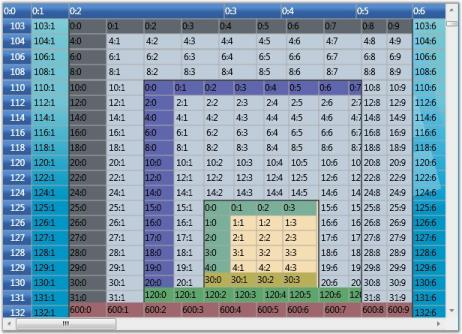Nested Grid-Rows tied to the Parent Grid Rows

Nested Grid Inside a Covered Range with its Columns Tied to the Columns of the Parent Grid

In this case the grid will have its own unique row height but the column widths are shared with the parent grid. When scrolling through columns in the nested grid you also scroll the columns in the parent grid to keep them in sync. The nested grid will have no scrollbars. They are shared with the parent grid. When you resize columns they will also be resized in parent grid and vice versa.

#### Example

To specify shared column layout, use Shared option of GridNestedAxisLayout enum in the second parameter.

``````// Add the appropriate nested grid cell model.
GridCellNestedGridModel shareColumn = new GridCellNestedGridModel (GridNestedAxisLayout.Normal, GridNestedAxisLayout.Shared);
Model[60, 1].CellType = "ShareColumnLayoutGrid";
Model[60, 1].BorderMargins.Top = 0;
Model[60, 1].BorderMargins.Left = 0;
Model[60, 1].BorderMargins.Right = 0;
Model[60, 1].BorderMargins.Bottom = 0;
Model[60, 1].Background = SystemColors.InactiveCaptionBrush;
GridModel nestedGridWithSharedColumnsModel = GetNestedGridWithSharedColumnsModel();

// Creates a nested grid with shared column layout.
Model[60, 1].CellValue = nestedGridWithSharedColumnsModel;
CoveredCells.Add(new CoveredCellInfo(60, 1, 80, 1 + nestedGridWithSharedColumnsModel.ColumnCount - 1));

// Sets up a nested grid with column layout shared

private GridModel GetNestedGridWithSharedColumnsModel()
{
GridModel model = new GridModel();
Pen gridLinePen = new Pen(Brushes.DarkGray, 1);
gridLinePen.Freeze();
model.Options.AllowSelection = GridSelectionFlags.Cell;
model.ColumnCount = 10;
model.RowHeights.FooterLineCount = 1;
model.RowCount = 13;
model.RowHeights.DefaultLineSize = 30;
Color clr = Color.FromArgb(128, 0, 0, 0);
Color clr2 = Color.FromArgb(128, 128, 0, 0);
Brush footerBrush = new SolidColorBrush(clr2);
footerBrush.Freeze();

for (int n = 0; n < model.RowCount; n++)
{

for (int c = 0; c < model.ColumnCount; c++)
{
GridStyleInfo ci = new GridStyleInfo();
ci.CellType = "TextBox";
ci.CellValue = String.Format("{0}:{1}", n, c);
ci.BorderMargins.Top = gridLinePen.Thickness;
ci.BorderMargins.Left = gridLinePen.Thickness;
ci.BorderMargins.Right = gridLinePen.Thickness / 2;
ci.BorderMargins.Bottom = gridLinePen.Thickness / 2;
ci.Borders.Right = gridLinePen;
ci.Background = null;// Brushes.White;
ci.Borders.Bottom = gridLinePen;
model.Data[n, c] = ci.Store;

if (c == 0 || n == 0)
{
ci.CellType = "Static";
}

if (c == 3 || n == 3)
{
ci.CellType = "CheckBox";
ci.CellValue = false;
}

if (c == 4 || n == 4)
{
ci.CellType = "Static";
ci.CellValue = "Static";
}

if (n == model.RowCount - 1)
{
ci.CellType = "Static";
ci.Background = footerBrush;
}
}
}
model.SelectedCells = GridRangeInfo.Empty;
return model;
}``````

#### Output

The following output is generated using the code above.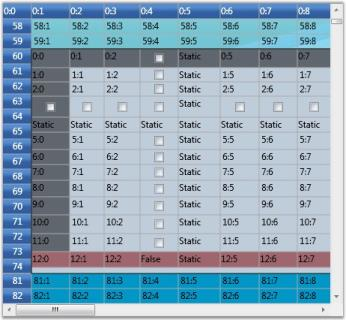Nested Grid-Columns tied to the Parent Grid Columns

Nested Grid Inside a Covered Range with its Rows and Columns Independent of Parent Grid

In this case, the nested grid maintains its own row heights and column widths. You can scroll through this grid without scrolling the parent grid. Resizing rows and columns in this grid will also not affect the parent grid.

#### Example

To make rows and columns independent of parent grid, the GridNestedAxisLayout enum must be set to Normal in both the parameters.

``````// Add Nested Grid cell model.
GridCellNestedGridModel gridModel = new GridCellNestedGridModel (GridNestedAxisLayout.Normal, GridNestedAxisLayout.Normal);
Model[6, 2].CellType = "Grid";

// Create a simple nested grid.
GridModel model = new GridModel();
model.Options.AllowSelection = GridSelectionFlags.Cell;
model.RowHeights.DefaultLineSize = 20;
model.RowCount = 20;
model.ColumnWidths.DefaultLineSize = 50;
model.ColumnCount = 8;
model.FrozenRows = 0;
model.FrozenColumns = 1;

for (int n = 0; n < model.RowCount; n++)
{

for (int c = 0; c < model.ColumnCount; c++)
{
GridStyleInfo ci = new GridStyleInfo();
ci.CellType = "TextBox";
ci.CellValue = String.Format("{0}:{1}", n, c);

//ci.Background =  transparentBlanchedAlmond;
model.Data[n, c] = ci.Store;
}
}
Model[6, 2].CellValue = model;``````

#### Output

The following output is generated using the code above.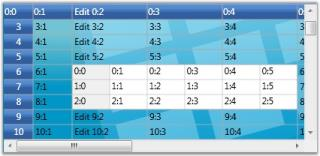Nested Grid-Rows and Columns Independent of Parent Grid

NOTE

For complete code, please refer to the following browser sample. …\My Documents\Syncfusion\EssentialStudio<Version Number>\Grid.WPF\Samples\3.5\WindowsSamples\Cell Types\Nested Grid Demo

## Formula Cells

Setting the CellType of a cell to a FormulaCell will allow you to enter algebraic expressions using formulas and cell references. Cell references are entries such as A11 for column A row 11 or BA3 for column BA row 3. A formula is a defined calculation from the Formula Library which, is included with Essential Grid. This Formula Library is extensible and allows you to add additional formulas.

This section comprises the following topics:

### Defining a FormulaCell

You can use FormulaCells for every cell in a grid or for just a few cells. Even if you assign a CellType FormulaCell to every cell in a grid, the default behavior is to treat such cells as text box cells unless you start the cell entry with an equal sign. If the cell value starts with an equal sign then, the cell is considered as a formula cell and its contents are treated as such.

To make all cells present in a grid as potential formula cells, you will have to set the CellType of the standard BaseStyle to a FormulaCell using the following code.

``````// Set up a Formula Cell.
this.gridControl1.BaseStylesMap["Standard"].StyleInfo.CellType = "FormulaCell";``````
``````'  Set up a Formula Cell.
Me.GridControl1.BaseStylesMap("Standard"). StyleInfo.CellType = "FormulaCell"``````

### Using the Formula Library

Essential Grid’s Formula Library contains the mathematical functions that are available in the .NET FrameWork’s System.Math class. In addition, there are Sum and Avg members. For a complete list of these library functions, please see the Class Reference for GridFormulaEngine. You can also add additional functions to this library using your own code.Sample Formula Library Usage

In the above image, cell A2 has a formula that uses four different library functions: Sqrt, Pow, Cos, and Sin.

### Supported Arithmetic Operators and Calculation Precedence

The current formula support will allow you to enter well-formed parenthetical algebraic expressions using operators and operands. The nine supported operators are shown in the precedence table given below, with operators on the same level being calculated as encountered when the expression is scanned from left to right.

Operations Symbols Calculation Precedence
Multiplication, Division / * 1st
Less Than, Greater Than, Equal, Less Than Or Equal, Greater Than Or Equal, Not Equal < > = <= >= <> 3rd

The supported operands include those listed in the table given below. An operand by itself is also a well-formed algebraic expression that can serve as an entire formula in a cell.

Operand

Operand Examples
number 532.1, -10.2, or 18.
cell reference A12, BB1010, or Q18.
library formula with valid arguments Abs(E14), Cos(-3.14), or Sum(A1:A14).
any well formed algebraic expression E1+E2, Cos(2)<A4, or Abs(A1-A5).

Within a formula cell, a case is ignored. So, a1 is the same as A1, and Cos(3) is the same as COS(3).

### Adding Formulas to the Formula Library

Here are the steps that are required to add a function to the Function Library.

1. First, define a method that has this signature.
``````// Define a method whose name is the FormulaName.

public string MyLibraryFormulaName(string args)``````
``````'Define a method whose name is the FormulaName.

Public Function MyLibraryFormulaName(ByVal args As String) As String``````

Here MyLibraryFormulaName must be a name that has not been already used in the Function Library and must include only letters and digits. If you want to replace an existing formula with a formula of the same name, first remove the existing formula before adding the new formula. Use the GridFormulaEngine.RemoveFunction method to remove a formula.

Then, write an implementation for your method. Here we use code to implement a function that will sum only positive numbers that are passed in as either a range like A1:A5 and/or a list such as A1, A4, A10. The code uses the FormulaEngine helper method to extract these values from the cells. The GetCellsFromArgs method will return an array of cells from a range such as A1:A5, and GetValueFromArgs method will take cells such as A3 and return a value such as 123.3.

``````// Implement Your Method.

public string ComputeSumPosNums(string args)
{
GridFormulaCellModel model = this.gridControl1.CellModels["FormulaCell"] as GridFormulaCellModel;

if(model != null)
{
GridFormulaEngine engine = model.Engine;
double sum = 0d;
double d;
string s1;

// Loop through any arguments and sum up the positive values.

foreach(string r in args.Split(new char[]{','}))
{
// Cell range

if(r.IndexOf(':') > -1)
{

foreach(string s in engine.GetCellsFromArgs(r))
{
// s is a cell line a21 or c3, and so on.

try
{
s1 = engine.GetValueFromArg(s);
}

catch(Exception ex)
{
return ex.Message;
}

if(s1 != "")
{

if(double.TryParse(s1, NumberStyles.Number, null, out d)
&& d > 0)
{
sum += d;
}
}
}
}

else
{

try
{
s1 = engine.GetValueFromArg(r);
}

catch(Exception ex)
{
return ex.Message;
}

if(s1 != "")
{

if(double.TryParse(s1, NumberStyles.Number, null, out d) && d > 0)
{
sum += d;
}
}
}
}
return sum.ToString();
}
return "";
}``````
``````' Implement Your Method.

Public Function ComputeSumPosNums(args As String) As String
Dim model As GridFormulaCellModel = Me.gridControl1.CellModels("FormulaCell")
If Not (model Is Nothing) Then
Dim engine As GridFormulaEngine = model.Engine
Dim sum As Double = 0.0
Dim d As Double
Dim s1 As String

' Loop through any arguments and sum up the positive values.
Dim r As String

For Each r In args.Split(New Char() {","c})

' Cell range

If r.IndexOf(":"c) > -1 Then
Dim s As String

For Each s In engine.GetCellsFromArgs(r)

' s is a cell line a21 or c3, and so on.

Try
s1 = engine.GetValueFromArg(s)

Catch ex As Exception
Return ex.Message
End Try

If s1 <> "" Then

If Double.TryParse(s1, NumberStyles.Number, Nothing, d) And d > 0 Then
sum += d
End If
End If
Next s
Else

Try
s1 = engine.GetValueFromArg(r)

Catch ex As Exception
Return ex.Message
End Try

If s1 <> "" Then

If Double.TryParse(s1, NumberStyles.Number, Nothing, d) And d > 0 Then
sum += d
End If
End If
End If
Next r
Return sum.ToString()
End If
Return ""

' SumPosNums
End Function``````

The last step is to actually add your formula to the library. You should do this after the grid has been created, say in a Form.Load event handler.

``````GridFormulaCellModel cellModel = this.gridControl1.CellModels["FormulaCell"] as GridFormulaCellModel;

// Add a formula named SumPosNums to the Library.
``````Dim cellModel As GridFormulaCellModel = Me.gridControl1.CellModels("FormulaCell")

' Add a formula named SumPosNums to the Library.

### Function Reference Section

The Syncfusion Grid control supports 370 formulas under various categories including math, statistical, logical, engineering, information, date, time, text, web, financial, lookup, and database functions.

### Math & Trigonometry functions

Name Description Syntax
ABS Returns the number without its sign. ABS(number)
ACOS Returns the arccosine, or inverse cosine, of a number. The returned angle is given in radians in the range 0 (zero) to pi. ACOS(number)number must be in the range of -1 to 1.
ACOSH Returns the inverse hyperbolic cosine of a number.The inverse hyperbolic cosine is the value whose hyperbolic cosine is number. ACOSH(number)number must be a real number.
ACOT Returns the principal value of the arc cotangent, or inverse cotangent, of a number. ACOT(number)number must be a real number.
ACOTH Returns the inverse hyperbolic cotangent of a number. ACOTH(number)number must be greater than 1.
ARABIC Converts a Roman numeral to an Arabic numeral. ARABIC( text )
ASIN Returns the arcsine, or inverse sine, of a number.The returned angle is given in radians in the range -pi/2 to pi/2. ASIN(number)number must be in the range of -1 to 1.
ASINH Returns the inverse hyperbolic sine of a number. ASINH(number)number must be a real number.
ATAN Returns the arctangent, or inverse tangent, of a number.The returned angle is given in radians in the range -pi/2 to pi/2. ATAN(number)
ATAN2 Returns the arctangent, or inverse tangent, of the specified x- and y-coordinates. ATAN2(x_num, y_num)
ATANH Returns the inverse hyperbolic tangent of a number. ATANH(number)number must be in the range of -1 and 1.
BASE Converts a number into a text representation with the given radix. BASE(Number, Radix, [Min_length])Number must be an integer greater than or equal to 0 and less than 2^53.Radix must be an integer greater than or equal to 2 and less than or equal to 36.Min_Length must be an integer greater than or equal to 0. (Optional)
CEILING Returns number rounded up to the nearest multiple of significance. CEILING(number, significance)
CEILING.MATH Rounds a number up to the nearest integer or to the nearest multiple of significance. CEILING(number, [significance], [mode])number must be less than 9.99E+307 and greater than -2.229E-308.Significance: The multiple to which the number is to be rounded. (Optional)Mode: For negative numbers, controls whether number is rounded toward or away from zero. (Optional)
COMBIN Returns the number of combinations for a given number of items. COMBIN(number, number_chosen)
COMBINA Returns the number of combinations (with repetitions) for a given number of items. COMBINA(number, number_chosen)
COS Returns the cosine of the given angle. COS(number)
COSH Returns the hyperbolic cosine of a number. COSH(number)
COT Returns the cotangent of an angle specified in radians. COT(number)
COTH Returns the hyperbolic cotangent of a hyperbolic angle. COTH(number)
CSC Returns the cosecant of an angle specified in radians. CSC(number)
CSCH Returns the hyperbolic cosecant of an angle specified in radians. CSCH(number)
DECIMAL Converts a text representation of a number in a given base into a decimal number. DECIMAL(text, radix)Radix must be an integer.
DEGREES Converts radians into degrees. DEGREES(angle)
EVEN Returns number rounded up to the nearest even integer. EVEN(number)
EXP Returns e raised to the power of number. The constant e equals 2.71828182845904, the base of the natural logarithm. EXP(number)
FACT Returns the factorial of a number. FACT(number)
FACTDOUBLE Returns the double factorial of a number. FACTDOUBLE(number)
FLOOR Rounds number down, toward zero, to the nearest multiple of significance. FLOOR(number, significance)
GCD Returns the greatest common divisor of two or more integers. GCD(number1, [number2], ...)
INT Rounds a number down to the nearest integer. INT(number)
LCM Returns the least common multiple of integers. LCM(number1, [number2], ...)
LN Returns the natural logarithm of a number. LN(number)
LOG Returns the logarithm of a number to the base you specify. LOG(number, [base])Number must be a positive real number.Base: Optional, if base is omitted it is assumed to be 10.
LOG10 Returns the base 10 logarithm of a number. LOG10(number)
MDETERM Returns the matrix determinant of an array. MDETERM(array)Array is a numeric array with an equal number of rows and columns.
MINVERSE Returns the inverse matrix for the matrix stored in an array. MINVERSE(array)Array is a numeric array with an equal number of rows and columns.
MMULT Returns the matrix product of two arrays. MMULT(array1, array2)
MOD Returns the remainder after number is divided by divisor. The result has the same sign as divisor. MOD(number, divisor)
MROUND Returns a number rounded to the desired multiple. MROUND(number, multiple)
MULTINOMIAL Returns the ratio of the factorial of a sum of values to the product of factorials. MULTINOMIAL(number1, [number2], ...)
MUNIT Returns the unit matrix for the specified dimension. MUNIT(dimension)Dimension must be an integer greater than 0.
ODD Returns number rounded up to the nearest odd integer. ODD(number)
PI Returns the number 3.14159265358979 PI()
POW Returns the result of a number raised to a power. POW(number, power)
POWER Returns the result of a number raised to a power. POWER(number, power)
PRODUCT Multiplies all of the numbers given as arguments and returns the product. PRODUCT(number1, [number2], ...)
QUOTIENT Returns the integer portion of a division. QUOTIENT(numerator, denominator)
ROMAN Converts an Arabic numeral to a Roman numeral as text. ROMAN(number, [form])Number is an Arabic numeral you want converted.Form: Optional. A number specifying the type of Roman numeral you want
ROUND Rounds a number to a specified number of digits. ROUND(number, num_digits)
ROUNDDOWN Rounds a number down, toward zero. ROUNDDOWN(number, num_digits)
ROUNDUP Rounds a number up, away from zero. ROUNDUP(number, num_digits)
SEC Returns the secant of an angle. SEC(number)
SECH Returns the hyperbolic secant of an angle. SECH(number)
SIGN Determines the sign of a number. Returns 1 if the number is positive, zero (0) if the number is 0, and -1 if the number is negative. SIGN(number)Number must be a real number.
SIN Returns the sine of the given angle. SIN(number)
SINH Returns the hyperbolic sine of a number. SINH(number)Number must be a real number.
SQRT Returns a positive square root. SQRT(number)
SQRTPI Returns the square root of (number * pi). SQRTPI(number)
SUBTOTAL Returns a subtotal in a list or database. SUBTOTAL(function_num,ref1,[ref2],...)function_num specifies which function to use in calculating subtotals.

Function_num
Function
1 101 AVERAGE
2 102 COUNT
3 103 COUNTA
4 104 MAX
5 105 MIN
6 106 PRODUCT
7 107 STDEV
8 108 STDEVP
9 109 SUM
10 110 VAR
11 111 VARP
The function_num 1-11 includes hidden cell values, and the 101-111 ignores the hidden values. Ref1: NamedRange or References Ref2: Optional , named range or cell references.
SUM Adds all the numbers that have been specified as arguments. SUM(number1,[number2],...)
SUMIFS Adds the cells in a range that meet multiple criteria. SUMIFS(sum_range,criteria_range1, criteria1, [criteria_range2, criteria2], ...)Sum_range: One or more cells to sum, including numbers or names, ranges, or cell references that contain numbers. Blank and text values are ignored.Criteria_range1: The first range in which to evaluate the associated criteria.Criteria1: The criteria in the form of a number, expression etc.Criteria_range2 , Criteria2: Optional.
SUMPRODUCT Multiplies corresponding components in the given arrays, and returns the sum of those products. SUMPRODUCT(array1, [array2], [array3], ...)Array1: The first array argument whose components you want to multiply and then add.Array2, array3,... Optional.
SUMSQ Returns the sum of the squares of the arguments. SUMSQ(number1, [number2], ...)
SUMX2MY2 Returns the sum of the difference of squares of corresponding values in two arrays. SUMX2MY2(array_x, array_y)
SUMX2PY2 Returns the sum of the sum of squares of corresponding values in two arrays. SUMX2PY2(array_x, array_y)
SUMXMY2 Returns the sum of squares of differences of corresponding values in two arrays. SUMXMY2(array_x, array_y)
TAN Returns the tangent of the given angle. TAN(number)
TANH Returns the hyperbolic tangent of a number. TANH(number)
TRUNC Truncates a number to an integer by removing the fractional part of the number. TRUNC(number, [num_digits])
TRUNCATE Truncates a number to an integer by removing the fractional part of the number. TRUNCATE(number, [num_digits])

### Statistical functions

Name Description Syntax
AVEDEV Returns the average of the absolute deviations of data points from their mean. AVEDEV(number1, [number2], ...)
AVERAGE Returns the average (arithmetic mean) of the arguments. AVERAGE(number1, [number2], ...)
AVERAGEA Calculates the average (arithmetic mean) of the values in the list of arguments. AVERAGEA(value1, [value2], ...)
AVE Equivalent to Average. Added for compatibility with older versions. AVE(number1, [number2], ...)
BINOM.DIST Returns the individual term binomial distribution probability. BINOM.DIST(number_s,trials,probability_s,cumulative)Number_s: The number of successes in trials.Trials: The number of independent trials.Probability_s: The probability of success on each trial.Cumulative: A logical value (TRUE/FALSE)
BINOM.INV Returns the smallest value for which the cumulative binomial distribution is greater than or equal to a criterion value. BINOM.INV(trials,probability_s,alpha)Trials: The number of Bernoulli trials.Probability_s:  The probability of a success on each trial.Alpha: The criterion value.
BINOMDIST Equivalent to BINOM.DIST, added for compatibility with older versions. BINOMDIST(number_s,trials,probability_s,cumulative)
CHIDIST Equivalent to CHISQ.DIST, added for compatibility with older versions. CHIDIST(x,deg_freedom)
CHIINV Equivalent to CHISQ.INV, added for compatibility with older versions. CHIINV(probability,deg_freedom)
CHISQ.DIST.RT Returns the right-tail probability of the chi-squared distribution. CHISQ.DIST.RT(x,deg_freedom)X : The value at which you want to evaluate the distribution.Deg_freedom : The number of degrees of freedom.
CHISQ.INV Returns the inverse of the left-tail probability of the chi-squared distribution. CHISQ.INV(probability,deg_freedom)Probability: A probability associated with the chi-squared distribution.Deg_freedom: The number of degrees of freedom.
CHISQ.INV.RT Returns the inverse of the right-tail probability of the chi-squared distribution. CHISQ.INV.RT(probability,deg_freedom)Probability: A probability associated with the chi-squared distribution.Deg_freedom: The number of degrees of freedom.
CHISQ.TEST Returns the value from the chi-squared (χ2) CHISQ.TEST(actual_range,expected_range)Actual_range: The range of data that contains observations to test against expected values.Expected_range: The range of data that contains the ratio of the product of row totals and column totals to the grand total.
CHITEST Equivalent to CHISQ.TEST, added for compatibility with older versions. CHITEST(actual_range,expected_range)
CONFIDENCE Returns the confidence interval for a population mean, using a normal distribution. CONFIDENCE(alpha,standard_dev,size)Alpha: The significance level used to compute the confidence level. Standard_dev: The population standard deviation for the data range, it is assumed to be known.Size: The sample size.
CONFIDENCE.NORM Returns the confidence interval for a population mean, using a normal distribution. CONFIDENCE.NORM(alpha,standard_dev,size)Alpha: The significance level used to compute the confidence level. Standard_dev: The population standard deviation for the data range, it is assumed to be known.Size: The sample size.
CONFIDENCE.T Returns the confidence interval for a population mean, using a student's t distribution. CONFIDENCE.T(alpha,standard_dev,size)
CORREL Returns the correlation coefficient of the Array1 and Array2 cell ranges. CORREL(array1, array2)Array1: A cell range of values.Array2: A second cell range of values.
COUNT Counts the number of cells that contain numbers. COUNT(value1, [value2], ...)Value1, value2: Cell Reference or Range
COUNTA Counts the number of cells that are not empty in a range. COUNTA(value1, [value2], ...)value1: The first argument representing the values that you want to count.value2: Additional arguments representing the values that you want to count. (Optional)
COUNTBLANK Counts the empty cells in a specified range of cells. COUNTBLANK(range)
COUNTIF Counts the number of cells within a range that meet a single criterion that you specify. COUNTIF(range, criteria)range: One or more cells to count.criteria: A number, expression, cell reference, or text string.
COVAR Returns covariance, the average of the products of deviations for each data point pair in two data sets. COVAR(array1,array2)Array1: The first cell range of integers.Array2: The second cell range of integers.
COVARIANCE.P Returns population covariance, the average of the products of deviations for each data point pair in two data sets. COVARIANCE.P(array1,array2)Array1: The first cell range of integers.Array2: The second cell range of integers.
COVARIANCE.S Returns the sample covariance, the average of the products of deviations for each data point pair in two data sets. COVARIANCE.S(array1,array2)Array1: The first cell range of integers.Array2: The second cell range of integers.
CRITBINOM Equivalent to BINOM.INV, added for compatibility with older versions. CRITBINOM(trials,probability_s,alpha)
DEVSQ Returns the sum of squares of deviations of data points from their sample mean. DEVSQ(number1, [number2], ...)
EXPON.DIST Returns the exponential distribution. EXPON.DIST(x,lambda,cumulative)X: The value of the function.Lambda: The parameter value.Cumulative: A logical value. If cumulative is TRUE, EXPON.DIST returns the cumulative distribution function; if FALSE, it returns the probability density function.
EXPONDIST Equivalent to EXPON.DIST, added for Compatibility with older versions. EXPONDIST(x,lambda,cumulative)
F.DIST Returns the F probability distribution. F.DIST(x,deg_freedom1,deg_freedom2,cumulative)X: The value at which to evaluate the function.Deg_freedom1: The numerator degrees of freedom.Deg_freedom2: The denominator degrees of freedom.Cumulative: A logical value. If cumulative is TRUE, F.DIST returns the cumulative distribution function; if FALSE, it returns the probability density function.
F.DIST.RT Returns the (right-tailed) F probability distribution for two data sets. F.DIST.RT(x,deg_freedom1,deg_freedom2)X :The value at which to evaluate the function.Deg_freedom1: The numerator degrees of freedom.Deg_freedom2: The denominator degrees of freedom.
F.INV.RT Returns the inverse of the (right-tailed) F probability distribution. F.INV.RT(probability,deg_freedom1,deg_freedom2)Probability: A probability associated with the F cumulative distribution.Deg_freedom1: The numerator degrees of freedom.Deg_freedom2: The denominator degrees of freedom.
FDIST Equivalent to F.DIST, added for compatibility with older versions. FDIST(x,deg_freedom1,deg_freedom2)
FINV Equivalent to F.INV, added for compatibility with older versions. FINV(probability,deg_freedom1,deg_freedom2)
FISHER Returns the Fisher transformation at x. FISHER(x)X: A numeric value for which you want the transformation.
FISHERINV Returns the inverse of the Fisher transformation. FISHERINV(y)Y: The value for which you want to perform the inverse of the transformation.
FORECAST Calculates, or predicts, a future value using existing values. FORECAST(x, known_y's, known_x's)X: The data point for which you want to predict a value.Known_y's: The dependent array or range of data.Known_x's: The independent array or range of data.
GAMMA.DIST Returns the gamma distribution. GAMMA.DIST(x,alpha,beta,cumulative)X: The value at which you want to evaluate the distribution.Alpha: A parameter to the distribution.Beta: A parameter to the distribution. Cumulative: If cumulative is TRUE, GAMMA.DIST returns the cumulative distribution function; if FALSE, it returns the probability density function.
GAMMA.INV Returns the inverse of the gamma cumulative distribution. GAMMA.INV(probability,alpha,beta)Probability: The probability associated with the gamma distribution.Alpha: A parameter to the distribution.Beta: A parameter to the distribution. If beta = 1, GAMMA.INV returns the standard gamma distribution.
GAMMAINV Equivalent to GAMMA.INV, added for compatibility with older versions. GAMMAINV(probability,alpha,beta)
GAMMALN Returns the natural logarithm of the gamma function, Γ(x). GAMMALN(x)X: The value for which you want to calculate GAMMALN.
GAMMALN.PRECISE Returns the natural logarithm of the gamma function, Γ(x). GAMMALN.PRECISE(x)X: The value for which you want to calculate GAMMALN.PRECISE.
GEOMEAN Returns the geometric mean of an array or range of positive data. GEOMEAN(number1, [number2], ...)
HARMEAN Returns the harmonic mean of a data set. HARMEAN(number1, [number2], ...)
HYPGEOM.DIST Returns the hypergeometric distribution. HYPGEOM.DIST(sample_s,number_sample,population_s,number_pop, ,cumulative)Sample_s: The number of successes in the sample.Number_sample: The size of the sample.Population_s: The number of successes in the population.Number_pop: The population size.Cumulative: If cumulative is TRUE, then HYPGEOM.DIST returns the cumulative distribution function; if FALSE, it returns the probability mass function.
HYPGEOMDIST Equivalent to HYPGEOM.DIST, added for compatibility with older versions. HYPGEOMDIST(sample_s,number_sample,population_s,number_pop)
INTERCEPT Calculates the point at which a line will intersect the y-axis by using existing x-values and y-values. INTERCEPT(known_y's, known_x's)Known_y's: The dependent set of observations or data.Known_x's: The independent set of observations or data.
KURT Returns the kurtosis of a data set. KURT(number1, [number2], ...)
LARGE Returns the k-th largest value in a data set. LARGE(array, k)Array: The array or range of data .K: The position in the array or cell range of data to return
LOGNORM.DIST Returns the log-normal distribution of x. LOGNORM.DIST(x,mean,standard_dev,cumulative)X: The value at which to evaluate the function.Mean: The mean of ln(x).Standard_dev: The standard deviation of ln(x).Cumulative: If cumulative is TRUE, LOGNORM.DIST returns the cumulative distribution function; if FALSE, it returns the probability density function.
LOGNORM.INV Returns the inverse of the log-normal cumulative distribution function of x. LOGNORM.INV(probability, mean, standard_dev)Probability: A probability associated with the log-normal distribution.Mean: The mean of ln(x).Standard_dev: The standard deviation of ln(x).
LOGNORMDIST Equivalent to LOGNORM.DIST, added for Compatibility with older versions. LOGNORMDIST(x,mean,standard_dev)
MAX Returns the largest value in a set of values. MAX(number1, [number2], ...)
MAXA Returns the largest value in the list of arguments. MAXA(value1,[value2],...)
MEDIAN Returns the median of the given numbers. MEDIAN(number1, [number2], ...)
MIN Returns the smallest value in a set of values. MIN(number1, [number2], ...)
MINA Returns the smallest value in the list of arguments. MINA(value1, [value2], ...)
MODE Returns the most frequently occurring, or repetitive, value in an array or range of data. MODE(number1,[number2],...)
NEGBINOM.DIST Returns the negative binomial distribution. NEGBINOM.DIST(number_f,number_s,probability_s,cumulative)Number_f: The number of failures.Number_s: The threshold number of successes.Probability_s: The probability of a success.Cumulative: If cumulative is TRUE, NEGBINOM.DIST returns the cumulative distribution function; if FALSE, it returns the probability density function.
NEGBINOMDIST Equivalent to NEGBINOM.DIST, added for compatibility with old versions. NEGBINOMDIST(number_f,number_s,probability_s)
NORM.DIST Returns the normal distribution for the specified mean and standard deviation. NORM.DIST(x,mean,standard_dev,cumulative)X: The value for which you want the distribution.Mean: The arithmetic mean of the distribution.Standard_dev: The standard deviation of the distribution.Cumulative: If cumulative is TRUE, NORM.DIST returns the cumulative distribution function; if FALSE, it returns the probability mass function.
NORM.INV Returns the inverse of the normal cumulative distribution for the specified mean and standard deviation. NORM.INV(probability,mean,standard_dev)Probability: A probability corresponding to the normal distribution.Mean: The arithmetic mean of the distribution.Standard_dev: The standard deviation of the distribution.
NORM.S.DIST Returns the standard normal distribution. NORM.S.DIST(z,cumulative)Z: The value for which you want the distribution.Cumulative: If cumulative is TRUE, NORMS.DIST returns the cumulative distribution function; if FALSE, it returns the probability mass function.
NORM.S.INV Returns the inverse of the standard normal cumulative distribution. NORM.S.INV(probability)Probability: A probability corresponding to the normal distribution.
NORMDIST Equivalent to NORM.DIST, added for compatibility with old versions. NORMDIST(x,mean,standard_dev,cumulative)
NORMINV Equivalent to NORM.INV, added for compatibility with old versions. NORMINV(probability,mean,standard_dev)
NORMSDIST Equivalent to NORM.S.DIST, added for compatibility with old versions. NORMSDIST(z,cumulative)
NORMSINV Equivalent to NORM.S.INV, added for compatibility with old versions. NORMSINV(z,cumulative)
PEARSON Returns the Pearson product moment correlation coefficient. PEARSON(array1, array2)Array1: A set of independent values.Array2: A set of dependent values.
PERCENTILE Returns the k-th percentile of values in a range. PERCENTILE(array,k)Array: The array or range of data that defines relative standing.K: The percentile value in the range of 0 to 1, inclusively.
PERCENTILE.EXC Returns the k-th percentile of values in a range, where k is in the range of 0 to 1, exclusively. PERCENTILE.EXC(array,k)Array: The array or range of data that defines relative standing.K: The percentile value in the range of 0 to 1, exclusively.
PERCENTILE.INC Returns the k-th percentile of values in a range, where k is in the range of 0 to 1, inclusively. PERCENTILE.INC(array,k)Array: The array or range of data that defines relative standing.K: The percentile value in the range 0 to 1, exclusively.
PERCENTRANK Returns the rank of a value in a data set as a percentage of the data set. PERCENTRANK(array,x,[significance])Array: The array or range of data with numeric values that defines relative standing.X: The value for which you want to know the rank.Significance: A value that identifies the number of significant digits for the returned percentage value.
PERCENTRANK.EXC Returns the rank of a value in a data set as a percentage (0 to 1, exclusively) of the data set. PERCENTRANK.EXC(array,x,[significance])Array: The array or range of data with numeric values that defines relative standing.X: The value for which you want to know the rank.Significance: A value that identifies the number of significant digits for the returned percentage value.
PERCENTRANK.INC Returns the rank of a value in a data set as a percentage (0 to1, inclusively) of the data set. PERCENTRANK.INC(array,x,[significance])Array: The array or range of data with numeric values that defines relative standing.X: The value for which you want to know the rank.Significance: A value that identifies the number of significant digits for the returned percentage value.
PERMUT Returns the number of permutations for a given number of objects that can be selected from number objects. PERMUT(number, number_chosen)Number: An integer that describes the number of objects.Number_chosen: An integer that describes the number of objects in each permutation.
PERMUTATIONA Returns the number of permutations for a given number of objects (with repetitions) that can be selected from the total objects. PERMUTATIONA(number, number-chosen)Number: An integer that describes the number of objects.Number_chosen: An integer that describes the number of objects in each permutation.
POISSON This function has been replaced with the POISSON.DIST function. This function is available for compatibility with older versions. POISSON(x,mean,cumulative)
POISSON.DIST Returns the Poisson distribution. POISSON.DIST(x,mean,cumulative)X: The number of events.Mean: The expected numeric value.Cumulative: If cumulative is TRUE, POISSON.DIST returns the cumulative Poisson distribution. If FALSE, it returns the Poisson probability mass function.
PROB Returns the probability that values in a range are between two limits. PROB(x_range, prob_range, [lower_limit], [upper_limit])X_range: The range of numeric values of x with which there are associated probabilities.Prob_range: A set of probabilities associated with values in x_range.Lower_limit: The lower limit on the value for which you want a probability.Upper_limit: The optional upper limit on the value for which you want a probability.
QUARTILE This function has been replaced with QUARTILE.EXC and QUARTILE.INC.However, this function is available for compatibility with older versions. QUARTILE(array,quart)
QUARTILE.EXC Returns the quartile of the data set, based on percentile values from 0 to1, exclusively. QUARTILE.EXC(array, quart)Array: The array or cell range of numeric values for which you want the quartile value.Quart: Indicates which value to return.
QUARTILE.INC Returns the quartile of a data set, based on percentile values from 0 to 1, inclusively. QUARTILE.INC(array,quart)Array: The array or cell range of numeric values for which you want the quartile value.Quart: Indicates which value to return.
RANK.AVG Returns the rank of a number in a list of numbers. RANK.AVG(number,ref,[order])Number: The number whose rank you want to find.Ref: An array of, or a reference to, a list of numbers. Non numeric values in Ref are ignored.Order: A number specifying how to rank number.
RANK.EQ Returns the rank of a number in a list of numbers. RANK.EQ(number,ref,[order])Number: The number whose rank you want to find.Ref: An array of, or a reference to, a list of numbers. Non numeric values in Ref are ignored.Order: A number specifying how to rank number.
RANK This function has been replaced with the RANK.AVG and RANK.EQ functions.This is available for compatibility with older versions. RANK(number,ref,[order])
RSQ Returns the square of the Pearson product moment correlation coefficient through data points in known_y's and known_x's. RSQ(known_y's,known_x's)Known_y's: An array or range of data points.Known_x's: An array or range of data points.
SKEW Returns the skewness of a distribution. SKEW(number1, [number2], ...)
SKEW.P Returns the skewness of a distribution based on a population. SKEW.P(number 1, [number 2],…)
SLOPE Returns the slope of the linear regression line through data points in known_y's and known_x's. SLOPE(known_y's, known_x's)Known_y's: An array or cell range of numeric dependent data points.Known_x's: The set of independent data points.
SMALL Returns the k-th smallest value in a data set. SMALL(array, k)Array: An array or range of numerical data for which you want to determine the k-th smallest value.K: The position (from the smallest) in the array or range of data to return.
STANDARDIZE Returns a normalized value from a distribution characterized by mean and standard_dev. STANDARDIZE(x, mean, standard_dev)X: The value you want to normalize.Mean: The arithmetic mean of the distribution.Standard_dev: The standard deviation of the distribution.
STDEV This function has been replaced with the STDEV.S function.This function is available for compatibility with older versions. STDEV(number1,[number2],...)
STDEV.P Calculates standard deviation based on the entire population given as arguments. STDEV.P(number1,[number2],...)
STDEV.S Estimates standard deviation based on a sample (ignores logical values and text in the sample). STDEV.S(number1,[number2],...)
STDEVA Estimates standard deviation based on a sample. STDEVA(value1, [value2], ...)
STDEVP This function has been replaced with the STDEV.P function. It is available for compatibility with older versions. STDEVP(number1,[number2],...)
STDEVPA Calculates standard deviation based on the entire population given as arguments, including text and logical values. STDEVPA(value1, [value2], ...)
STEYX Returns the standard error of the predicted y-value for each x in the regression. STEYX(known_y's, known_x's)Known_y's: An array or range of dependent data points.Known_x's: An array or range of independent data points.
T.INV Returns the left-tail inverse of the Student's t-distribution. T.INV(probability,deg_freedom)Probability: The probability associated with the Student's t-distribution.Deg_freedom: The number of degrees of freedom with which to characterize the distribution.
TRIMMEAN Returns the mean of the interior of a data set. TRIMMEAN(array, percent)Array: The array or range of values to trim and average.Percent: The fractional number of data points to exclude from the calculation.
VAR This function has been replaced with the VAR.S function.This is available for compatibility with older versions. VAR(number1,[number2],...)
VARA Estimates variance based on a sample. VARA(value1, [value2], ...)
VARP This function has been replaced with the VAR.P function.This is available for compatibility with older versions. VARP(number1,[number2],...)
VARPA Calculates the variance based on the entire population. VARPA(value1, [value2], ...)
WEIBULL This function has been replaced with the WEIBULL.DIST function.This is available for compatibility with older versions. WEIBULL(x,alpha,beta,cumulative)
WEIBULL.DIST Returns the Weibull distribution. WEIBULL.DIST(x,alpha,beta,cumulative)X: The value at which to evaluate the function.Alpha: A parameter for the distribution.Beta: A parameter for the distribution.Cumulative: Determines the form of the function.
Z.TEST Returns the one-tailed P-value of a z-test. Z.TEST(array,x,[sigma])Array: The array or range of data against which to test x.x: The value to test.Sigma: The population (known) standard deviation.
ZTEST This function has been replaced with the Z.TEST function.This is available for compatibility with older versions. ZTEST(array,x,[sigma])

#### Logical functions

Name Description Syntax
AND Returns TRUE if all of its arguments evaluate to TRUE.Returns FALSE if one or more arguments evaluate to FALSE. AND(logical1, [logical2], ...)logical1: The first condition that you want to test that can evaluate to either TRUE or FALSE.logical2: Additional conditions that you want to test that can evaluate to either TRUE or FALSE, up to a maximum of 255 conditions. (Optional)
IF The IF function returns one value if a specified condition evaluates to TRUE, and another value if that condition evaluates to FALSE. IF(logical_test,[value_if_true], [value_if_false])Logical_test: Any value or expression that can be evaluated to TRUE or FALSE.Value_if_true: The value that you want to be returned if the logical_test argument evaluates to TRUE. (Optional)Value_if_false : The value that you want to be returned if the logical_test argument evaluates to FALSE. (Optional)
IFERROR Returns a value you specify if a formula evaluates to an error; otherwise, returns the result of the formula. IFERROR(value, value_if_error)Value: The argument that is checked for an error.Value_if_error: The value to return if the formula evaluates to an error.
NOT Reverses the value of its argument. Use NOT when you want to make sure a value is not equal to one particular value. NOT(logical)Logical: A value or expression that can be evaluated to TRUE or FALSE.
OR Returns TRUE if any argument is TRUE; returns FALSE if all arguments are FALSE. OR(logical1, [logical2], ...)Logical1, logical2: 1 to 255 conditions you want to test that can be either TRUE or FALSE.
FALSE Returns the logical value FALSE. FALSE()
TRUE Returns the logical value TRUE. TRUE()

### Engineering functions

Name Description Syntax
BESSELI Returns the modified Bessel function. BESSELI(X, N)X: The value at which to evaluate the function.N: The order of the Bessel function. If n is not an integer, it is truncated.
BESSELJ Returns the Bessel function. BESSELJ(X, N)X: The value at which to evaluate the function.N: The order of the Bessel function. If n is not an integer, it is truncated.
BESSELK Returns the modified Bessel function, which is equivalent to the Bessel functions evaluated for purely imaginary arguments. BESSELK(X, N)X: The value at which to evaluate the function.N: The order of the function. If n is not an integer, it is truncated.
BESSELY Returns the Bessel function, which is also called the Weber function or the Neumann function. BESSELY(X, N)X: The value at which to evaluate the function.N: The order of the function. If n is not an integer, it is truncated.
BIN2DEC Converts a binary number to decimal. BIN2DEC(number)Number: The binary number you want to convert.
BIN2HEX Converts a binary number to hexadecimal. BIN2HEX(number, [places])Number: The binary number you want to convert.Places: The number of characters to use.
BIN2OCT Converts a binary number to octal. BIN2OCT(number, [places])Number: The binary number you want to convert.Places: The number of characters to use.
BITAND Returns a bitwise 'AND' of two numbers. BITAND( number1, number2)Number1,Number2: Must be in decimal form and greater than or equal to 0.
BITLSHIFT Returns a number shifted left by the specified number of bits. BITLSHIFT(number, shift_amount)Number: Number must be an integer greater than or equal to 0.Shift_amount: Shift_amount must be an integer.
BITOR Returns a bitwise 'OR' of two numbers. BITOR(number1, number2)Number1, Number2: Must be in decimal form and greater than or equal to 0.
BITRSHIFT Returns a number shifted right by the specified number of bits. BITRSHIFT(number, shift_amount)Number: Number must be an integer greater than or equal to 0.Shift_amount: Shift_amount must be an integer.
BITXOR Returns a bitwise 'XOR' of two numbers. BITXOR(number1, number2)Number1,Number2: Must be in decimal form and greater than or equal to 0.
COMPLEX Converts real and imaginary coefficients into a complex number of the form x + yi or x + yj. COMPLEX(real_num, i_num, [suffix])Real_num: The real coefficient of the complex number.I_num: The imaginary coefficient of the complex number.Suffix: The suffix for the imaginary component of the complex number. If omitted, suffix is assumed to be "i".
CONVERT Converts a number from one measurement system to another. CONVERT(number,from_unit,to_unit)Number: the value in from_units to convert.From_unit: the units for number.To_unit: the units for the result.
DEC2BIN Converts a decimal number to binary. DEC2BIN(number, [places])Number: The decimal integer you want to convert.Places: The number of characters to use.
DEC2HEX Converts a decimal number to hexadecimal. DEC2HEX(number, [places])Number: The decimal integer you want to convert.Places: The number of characters to use.
DEC2OCT Converts a decimal number to octal. DEC2OCT(number, [places])Number: The decimal integer you want to convert.Places: The number of characters to use.
ERF Returns the error function integrated between lower_limit and upper_limit. ERF(lower_limit,[upper_limit])Lower_limit: The lower bound for integrating ERF.Upper_limit: The upper bound for integrating ERF. (Optional)
ERF.PRECISE Returns the error function. ERF.PRECISE(x)X: The lower bound for integrating ERF.PRECISE.
ERFC.PRECISE Returns the complementary ERF function integrated between x and infinity. ERFC.PRECISE(x)X: The lower bound for integrating ERFC.PRECISE.
GESTEP Returns 1 if number ≥ step; returns 0 (zero) otherwise. GESTEP(number, [step])Number: The value to test against step.Step: The threshold value. If you omit a value for step, GESTEP uses zero. (Optional)
HEX2BIN Converts a hexadecimal number to binary. HEX2BIN(number, [places])Number: The hexadecimal number you want to convert.Places: The number of characters to use.
HEX2DEC Converts a hexadecimal number to decimal. HEX2DEC(number)Number: The hexadecimal number you want to convert.
HEX2OCT Converts a hexadecimal number to octal. HEX2OCT(number, [places])Number: The hexadecimal number you want to convert.Places: The number of characters to use.
IMABS Returns the absolute value of a complex number in x + yi or x + yj text format. IMABS(inumber)Inumber: A complex number for which you want the absolute value.
IMAGINARY Returns the imaginary coefficient of a complex number in x + yi or x + yj text format. IMAGINARY(inumber)Inumber: A complex number for which you want the imaginary coefficient.
IMARGUMENT Returns the argument Theta. IMARGUMENT(inumber)Inumber: A complex number for which you want the argument Theta.
IMCONJUGATE Returns the complex conjugate of a complex number in x + yi or x + yj text format. IMCONJUGATE(inumber)Inumber: A complex number for which you want the conjugate.
IMCOS Returns the cosine of a complex number in x + yi or x + yj text format. IMCOS(inumber)Inumber: A complex number for which you want the cosine.
IMCOSH Returns the hyperbolic cosine of a complex number in x+yi or x+yj text format. IMCOSH(inumber)Inumber: A complex number for which you want the hyperbolic cosine.
IMCOT Returns the cotangent of a complex number in x+yi or x+yj text format. IMCOT(inumber)Inumber: A complex number for which you want the cotangent.
IMCSC Returns the cosecant of a complex number in x+yi or x+yj text format. IMCSC(inumber)Inumber: A complex number for which you want the cosecant.
IMCSCH Returns the hyperbolic cosecant of a complex number in x+yi or x+yj text format. IMCSCH(inumber)Inumber: A complex number for which you want the hyperbolic cosecant.
IMDIV Returns the quotient of two complex numbers in x + yi or x + yj text format. IMDIV(inumber1, inumber2)Inumber1: The complex numerator or dividend.Inumber2: The complex denominator or divisor.
IMEXP Returns the exponential of a complex number in x + yi or x + yj text format. IMEXP(inumber)Inumber: A complex number for which you want the exponential.
IMLN Returns the natural logarithm of a complex number in x + yi or x + yj text format. IMLN(inumber)Inumber: A complex number for which you want the natural logarithm.
IMLOG10 Returns the common logarithm (base 10) of a complex number in x + yi or x + yj text format. IMLOG10(inumber)Inumber: A complex number for which you want the common logarithm.
IMLOG2 Returns the base-2 logarithm of a complex number in x + yi or x + yj text format. IMLOG2(inumber)Inumber: A complex number for which you want the base-2 logarithm.
IMPOWER Returns a complex number in x + yi or x + yj text format raised to a power. IMPOWER(inumber, number)Inumber: A complex number you want to raise to a power.Number: The power to which you want to raise the complex number.
IMPRODUCT Returns the product of 1 to 255 complex numbers in x + yi or x + yj text format. IMPRODUCT(inumber1, [inumber2], ...)
IMREAL Returns the real coefficient of a complex number in x + yi or x + yj text format. IMREAL(inumber)Inumber: A complex number for which you want the real coefficient.
IMSEC Returns the secant of a complex number in x+yi or x+yj text format. IMSEC(inumber)Inumber: A complex number for which you want the secant.
IMSECH Returns the hyperbolic secant of a complex number in x+yi or x+yj text format. IMSECH(inumber)Inumber: A complex number for which you want the hyperbolic secant.
IMSIN Returns the sine of a complex number in x + yi or x + yj text format. IMSIN(inumber)Inumber: A complex number for which you want the sine.
IMSINH Returns the hyperbolic sine of a complex number in x+yi or x+yj text format. IMSINH(inumber)Inumber: A complex number for which you want the hyperbolic sine.
IMSQRT Returns the square root of a complex number in x + yi or x + yj text format. IMSQRT(inumber)Inumber: A complex number for which you want the square root.
IMSUB Returns the difference of two complex numbers in x + yi or x + yj text format. IMSUB(inumber1, inumber2)Inumber1: The complex number from which to subtract inumber2.Inumber2: The complex number to subtract from inumber1.
IMSUM Returns the sum of two or more complex numbers in x + yi or x + yj text format. IMSUM(inumber1, [inumber2], ...)
IMTAN Returns the tangent of a complex number in x+yi or x+yj text format. IMTAN(inumber)Inumber: A complex number for which you want the tangent.
OCT2BIN Converts an octal number to binary. OCT2BIN(number, [places])Number: The octal number you want to convert.Places: The number of characters to use.
OCT2DEC Converts an octal number to decimal. OCT2DEC(number)Number: The octal number you want to convert.
OCT2HEX Converts an octal number to hexadecimal. OCT2HEX(number, [places])Number: The octal number you want to convert.Places: The number of characters to use.

### Information functions

Name Description Syntax
CELL Returns information about the formatting, location, or contents of a cell. CELL(info_type, [reference])Info_type: A text value that specifies what type of cell information you want to return.

 Info_type Returns 'col' Column Number of ref. 'color' 1 for negative values, otherwise 0. 'contents' Value of the upper left cell in ref. 'filename' Full path of the file that contains ref. 'format' Text value of the number format specified in the referenced cell. 'parenthesis' 1 if the cell is formatted with parentheses for positive or all values; otherwise returns 0. 'prefix' Label prefix of the cell. 'protect' 0 if the cell is not locked, otherwise 1. 'row' Row number of the cell in reference. 'type' B if the cell is empty.L if the cell contains a label.V if the cell contains a value. 'width' Column width of the ref cell.
ERROR.TYPE Returns a number corresponding to one of the error values in Microsoft Excel, or returns the #N/A error if no error exists. ERROR.TYPE(error_val)

 error_val Returns #NULL! 1 #DIV/0! 2 #VALUE! 3 #REF! 4 #NAME? 5 #NUM! 6 #N/A 7 #GETTING_DATA 8 Anything else #N/A
INFO Returns information about the current operating environment. INFO(type_text)

 Type_text Returns 'directory' Path of the current directory or folder. 'num file' Number of active worksheets in the open workbooks. 'origin' Returns the absolute cell reference of the top and leftmost cell visible in the window. 'OS version' Current operating system version, as text. 'recalc' Current recalculation mode; returns 'Automatic' or 'Manual'. 'release' Version of Microsoft Excel, as text. 'system' Name of the operating environment:Macintosh = "mac"Windows = "pcdos"
ISBLANK Returns TRUE if the cell is blank, otherwise returns FALSE. ISBLANK(value)
ISERR Returns TRUE if value refers to any error value except #N/A. ISERR(value)
ISERROR Returns TRUE if value refers to any error value (#N/A, #VALUE!, #REF!, #DIV/0!, #NUM!, #NAME?, or #NULL!). ISERROR(value)
ISEVEN Returns TRUE if number is even, or FALSE if number is odd. ISEVEN(number)
ISFORMULA Returns TRUE if referenced to a cell that contains a formula, otherwise FALSE. ISFORMULA(reference)
ISLOGICAL Returns TRUE if value refers to a logical value. ISLOGICAL(value)
ISNA Returns TRUE if value refers to the #N/A (value not available) error value. ISNA(value)
ISNONTEXT Returns TRUE if value refers to any item that is not text. ISNONTEXT(value)
ISNUMBER Returns TRUE if value refers to a number. ISNUMBER(value)
ISODD Returns TRUE if number is odd, or FALSE if number is even. ISODD(number)
ISTEXT Returns TRUE if value refers to text. ISTEXT(value)
N Returns a value converted to a number. N(value)
NA Returns the error value #N/A. #N/A is the error value that means "no value is available. NA( )
TYPE Returns the type of value. TYPE(value)

 value returns Number 1 Text 2 Logical value 4 Error value 16 Array (array: Used to build single formulas that produce multiple results or that operate on a group of arguments that are arranged in rows and columns. An array range shares a common formula; an array constant is a group of constants used as an argument.) 64

#### Date & Time functions

Date and Time functions

Name Description Syntax
DATE Returns the sequential serial number that represents a particular date. DATE(year,month,day)Year: The value of the year argument can include one to four digits.Month: A positive or negative integer representing the month of the year from 1 to 12 (January to December).Day: A positive or negative integer representing the day of the month from 1 to 31.
DATEVALUE Converts a date that is stored as text into a serial number that Excel recognizes as a date. DATEVALUE(date_text)Date_text: Text that represents a date in an Excel date format.
DAY Returns the day of a date, represented by a serial number. DAY(serial_number)Serial_number: The date of the day you are trying to find.
DAYS Returns the number of days between two dates. DAYS(end_date, start_date)Start_date and End_date are the two dates between which you want to know the number of days.
DAYS360 The DAYS360 function returns the number of days between two dates based on a 360-day year (twelve 30-day months). DAYS360(start_date,end_date,[method])Start_date, end_date: The two dates between which you want to know the number of days.Method: A logical value that specifies whether to use the U.S. or European method in the calculation.
EDATE Returns the serial number that represents the date that is the indicated number of months before or after a specified date. EDATE(start_date, months)Start_date: A date that represents the start date.Months: The number of months before or after start_date.
EOMONTH Returns the serial number for the last day of the month that is the indicated number of months before or after start_date. EOMONTH(start_date, months)Start_date: A date that represents the starting date.Months: The number of months before or after start_date.
HOUR Returns the hour of a time value. HOUR(serial_number)Serial_number: The time that contains the hour you want to find.
ISOWEEKNUM Returns number of the ISO week number of the year for a given date. ISOWEEKNUM(date)Date: Date is the date-time code used by Excel for date and time calculation.
MINUTE Returns the minutes of a time value. The minute is given as an integer, ranging from 0 to 59. MINUTE(serial_number)Serial_number: The time that contains the minute you want to find.
MONTH Returns the month of a date represented by a serial number. MONTH(serial_number)Serial_number: The date of the month you are trying to find.
NETWORKDAYS.INTL Returns the number of whole workdays between two dates using parameters to indicate which and how many days are weekend days. NETWORKDAYS.INTL (start_date, end_date, [weekend], [holidays])Start_date and end_date: The dates for which the difference is to be computed.Weekend is a weekend number or string that specifies when weekends occur.
NOW Returns the serial number of the current date and time. NOW()
SECOND Returns the seconds of a time value. SECOND(serial_number)Serial_number: The time that contains the seconds you want to find.
TIME Returns the decimal number for a particular time. TIME(hour, minute, second)Hour: A number from 0 (zero) to 32767 representing the hour.Minute: A number from 0 to 32767 representing the minute.Second: A number from 0 to 32767 representing the second.
TIMEVALUE Returns the decimal number of the time represented by a text string. TIMEVALUE(time_text)Time_text: A text string that represents a time in any of the time formats.
TODAY Returns the serial number of the current date. TODAY()
WEEKDAY Returns the day of the week corresponding to a date. WEEKDAY(serial_number,[return_type])Serial_number: A sequential number that represents the date you are trying to find.Return_type: A number that determines the type of return value. (Optional)
WEEKNUM Returns the week number of a specific date. WEEKNUM(serial_number,[return_type])Serial_number: A date within the week.Return_type: A number that determines on which day the week begins. The default is 1. (Optional)
WORKDAY Returns a number that represents a date that is the indicated number of working days before or after a date. WORKDAY(start_date, days, [holidays])Start_date: A date that represents the start date.Days: The number of non-weekend and non-holiday days before or after start_date.Holidays: An optional list of one or more dates to exclude from the working calendar, such as state and federal holidays and floating holidays.
WORKDAY.INTL Returns the serial number of the date before or after a specified number of workdays with custom weekend parameters. WORKDAY.INTL(start_date, days, [weekend], [holidays])Start_date: The start date, truncated to integer.Days: The number of workdays before or after the start_date.Weekend: Indicates the days of the week that are weekend days and are not considered working days. (Optional)
YEAR Returns the year corresponding to a date. YEAR(serial_number)Serial_number: The date of the year you want to find.
YEARFRAC Calculates the fraction of the year represented by the number of whole days between two dates. YEARFRAC(start_date, end_date, [basis])Start_date: A date that represents the start date.End_date: A date that represents the end date.Basis: The type of day count basis to use. (Optional)

 Basis Day count basis 0 or omitted US (NASD) 30/360 1 Actual/actual 2 Actual/360 3 Actual/365 4 European 30/360

### Financial functions

Financial functions

Name Description Syntax
CUMIPMT Returns the cumulative interest paid on a loan between start_period and end_period. CUMIPMT(rate, nper, pv, start_period, end_period, type)Rate: The interest rate.Nper: The total number of payment periods.Pv: The present value.Start_period: The first period in the calculation. End_period: The last period in the calculation.Type: The timing of the payment.

 Type Timing 0 (zero) Payment at the end of the period. 1 Payment at the beginning of the period.
CUMPRINC Returns the cumulative principal paid on a loan between start_period and end_period. CUMPRINC(rate, nper, pv, start_period, end_period, type)Rate: The interest rate.Nper: The total number of payment periods.Pv: The present value.Start_period: The first period in the calculation. End_period: The last period in the calculation.Type: The timing of the payment.

 Type Timing 0 (zero) Payment at the end of the period. 1 Payment at the beginning of the period.
DB Returns the depreciation of an asset for a specified period using the fixed-declining balance method. DB(cost, salvage, life, period, [month])Cost: The initial cost of the asset.Salvage: The value at the end of the depreciation Life: The number of periods over which the asset is being depreciated.Period: The period for which you want to calculate the depreciation. Period must use the same units as life.Month: The number of months in the first year. If month is omitted, it is assumed to be 12. (Optional)
DDB Returns the depreciation of an asset for a specified period using the double-declining balance method or some other method you specify. DDB(cost, salvage, life, period, [factor])Cost: The initial cost of the asset.Salvage: The value at the end of the depreciation This value can be 0.Life: The number of periods over which the asset is being depreciated.Period: The period for which you want to calculate the depreciation. Period must use the same units as life.Factor: The rate at which the balance declines. (Optional)
DISC Returns the discount rate for a security. DISC(settlement, maturity, pr, redemption, [basis])Settlement: The security's settlement date. Maturity: The security's maturity date. The maturity date is the date when the security expires.Pr: The security's price per \$100 face value.Redemption: The security's redemption value per \$100 face value.Basis: The type of day count basis to use. (Optional)
DOLLARDE Converts a dollar price expressed as an integer part and a fraction part, such as 1.02, into a dollar price expressed as a decimal number. DOLLARDE(fractional_dollar, fraction)Fractional_dollar: A number expressed as an integer part and a fraction part, separated by a decimal symbol.Fraction: The integer to use in the denominator of the fraction.
DOLLARFR Use DOLLARFR to convert decimal numbers to fractional dollar numbers, such as securities prices. DOLLARFR(decimal_dollar, fraction)Decimal_dollar: A decimal number.Fraction: The integer to use in the denominator of a fraction.
DURATION Returns the Macaulay duration for an assumed par value of \$100. DURATION(settlement, maturity, coupon, yield, frequency, [basis])Settlement: The security settlement date is the date after the issue date when the security is traded to the buyer.Maturity: The maturity date is the date when the security expires.Coupon: The security's annual coupon rate.Yield: The security's annual yield.Frequency: The number of coupon payments per year. For annual payments, frequency = 1; for semiannual, frequency = 2; for quarterly, frequency = 4.Basis: The type of day count basis to use. (Optional)

 Basis Day count basis 0 or omitted US (NASD) 30/360 1 Actual/actual 2 Actual/360 3 Actual/365 4 European 30/360
FV Returns the future value of an investment based on periodic, constant payments and a constant interest rate. FV(rate,nper,pmt,[pv],[type])Rate: The interest rate per period.Nper: The total number of payment periods in an annuity.Pmt: The payment made each period.Pv: The present value, or the lump-sum amount that a series of future payments is worth right now. If pv is omitted, it is assumed to be 0. (Optional)Type: The number 0 or 1, which indicates when payments are due. If type is omitted, it is assumed to be 0. (Optional)
FVSHEDULE Returns the future value of an initial principal after applying a series of compound interest rates. FVSCHEDULE(principal, schedule)Principal: The present value.Schedule: An array of interest rates to apply.
INTRATE Returns the interest rate for a fully invested security. INTRATE(settlement, maturity, investment, redemption, [basis])Settlement: The security settlement date is the date after the issue date when the security is traded to the buyer.Maturity: The maturity date is the date when the security expires.Investment: The amount invested in the security.Redemption: The amount to be received at maturity.Basis: The type of day count basis to use.

 Basis Day count basis 0 or omitted US (NASD) 30/360 1 Actual/actual 2 Actual/360 3 Actual/365 4 European 30/360
IPMT Returns the interest payment for a given period for an investment based on periodic, constant payments and a constant interest rate. IPMT(rate, per, nper, pv, [fv], [type])Rate: The interest rate per period.Per: The period for which you want to find the interest and must be in the range 1 to nper.Nper: The total number of payment periods in an annuity.Pv: The present value, or the lump-sum amount that a series of future payments is worth right now.Fv: The future value or a cash balance you want to attain after the last payment is made. If fv is omitted, it is assumed to be 0 (the future value of a loan, for example, is 0).Type: The number 0 or 1.

 Type Payment Due 0 At the end of the period. 1 At the beginning of the period.
IRR Returns the internal rate of return for a series of cash flows represented by the numbers in values. IRR(values, [guess])Values: An array or a reference to cellsGuess: A number that you guess is close to the result of IRR. (Optional)
ISPMT Calculates the interest paid during a specific period of an investment. This function is provided for compatibility with Lotus 1-2-3. ISPMT(rate, per, nper, pv)Rate: The interest rate for the investment.Per: The period for which you want to find the interest, and must be between 1 and nper.Nper: The total number of payment periods for the investment.Pv: The present value of the investment. For a loan, pv is the loan amount.
MIRR Returns the modified internal rate of return for a series of periodic cash flows. MIRR(values, finance_rate, reinvest_rate)Values: An array or a reference to cells that contain numbers.Finance_rate: The interest rate you pay on the money used in the cash flows.Reinvest_rate: The interest rate you receive on the cash flows as you reinvest them.
NPER Returns the number of periods for an investment based on periodic, constant payments and a constant interest rate. NPER(rate,pmt,pv,[fv],[type])Rate : The interest rate per period.Pmt : The payment made each period; Pv : The present value, or the lump-sum amount that a series of future payments is worth right now.Fv : The future value, or a cash balance you want to attain after the last payment is made. (Optional)Type : The number 0 or 1 and indicates when payments are due. (Optional)
NPV Calculates the net present value of an investment by using a discount rate and a series of future payments and income. NPV(rate,value1,[value2],...)Rate: The rate of discount over the length of one period.
PMT Calculates the payment for a loan based on constant payments and a constant interest rate. PMT(rate, nper, pv, [fv], [type])Rate: The interest rate for the loan.Nper: The total number of payments for the loan.Pv: The present value, or the total amount that a series of future payments is worth now.Fv: The future value, or a cash balance you want to attain after the last payment is made. Type: The number 0 (zero) or 1, it indicates when payments are due. (Optional)
PPMT Returns the payment on the principal for a given period for an investment based on periodic, constant payments and a constant interest rate. PPMT(rate, per, nper, pv, [fv], [type])Rate: The interest rate per period.Per: Specifies the period and must be in the range 1 to nper.Nper: The total number of payment periods in an annuity.Pv: The present value — the total amount that a series of future payments is worth now.Fv: The future value, or a cash balance you want to attain after the last payment is made. If fv is omitted, it is assumed to be 0 (zero), that is, the future value of a loan is 0.Type: The number 0 or 1, it indicates when payments are due. (Optional)
PV Returns the present value of an investment. The present value is the total amount that a series of future payments is worth now. PV(rate, nper, pmt, [fv], [type])Rate: The interest rate per period. Nper: The total number of payment periods in an annuity. Pmt: The payment made each period and cannot change over the life of the annuity. Fv: The future value, or a cash balance you want to attain after the last payment is made.Type: The number 0 or 1, it indicates when payments are due. (Optional)
RATE Returns the interest rate per period of an annuity. RATE(nper, pmt, pv, [fv], [type], [guess])Nper: The total number of payment periods in an annuity.Pmt: The payment made each period and cannot change over the life of the annuity. Pv: The present value — the total amount that a series of future payments is worth now.Fv: The future value, or a cash balance you want to attain after the last payment is made. Type: The number 0 or 1 and indicates when payments are due. (Optional)

 Type Payment Due 0 or omitted At the end of the period. 1 At the beginning of the period.
Guess: Your guess for what the rate will be. (Optional)
RRI Returns an equivalent interest rate for the growth of an investment. RRI(nper, pv, fv)Nper: Nper is the number of periods for the investment.Pv: Pv is the present value of the investment.Fv: Fv is the future value of the investment.
SLN Returns the straight-line depreciation of an asset for one period. SLN(cost, salvage, life)Cost: The initial cost of the asset.Salvage: The value at the end of the depreciation.Life: The number of periods over which the asset is depreciated.
SYD Returns the sum-of-years' digits depreciation of an asset for a specified period. SYD(cost, salvage, life, per)Cost: The initial cost of the asset.Salvage: The value at the end of the depreciation.Life: The number of periods over which the asset has depreciated.Per: The period, it must use the same units as life.
VDB Returns the depreciation of an asset for any specified period, including partial periods. VDB(cost, salvage, life, start_period, end_period, [factor], [no_switch])Cost: The initial cost of the asset.Salvage: The value at the end of the depreciation This value can be 0.Life: The number of periods over which the asset is depreciated.Start_period: The starting period for which you want to calculate the depreciation.End_period: The ending period for which you want to calculate the depreciation. Factor: The rate at which the balance declines. (Optional) No_switch: A logical value specifying whether to switch to straight-line depreciation when depreciation is greater than the declining balance calculation. (Optional)
XIRR Returns the internal rate of return for a schedule of cash flows that is not necessarily periodic. XIRR(values, dates, [guess])Values: A series of cash flows that corresponds to a schedule of payments in dates. Dates: A schedule of payment dates that corresponds to the cash flow paymentsGuess: A number that you guess is close to the result of XIRR. (Optional)

### Lookup & Reference functions

Lookup and Reference functions

Name Description Syntax
AREA Returns the number of areas in a reference. An area is a range of contiguous cells or a single cell. AREAS(reference)Reference: A reference to a cell or range of cells, it can refer to multiple areas.
COLUMN Returns the column number of the given cell reference. COLUMN([reference])Reference: The cell or range of cells for which you want to return the column number. (Optional)
COLUMNS Returns the number of columns in an array or reference. COLUMNS(array)Array: An array or array formula, or a reference to a range of cells for which you want the number of columns.
FORMULATEXT Returns a formula as a string. FORMULATEXT(reference)Reference: A reference to a cell or range of cells.
HLOOKUP Searches for a value in the top row of a table or an array of values, and then returns a value in the same column from a row you specify in the table or array. HLOOKUP(lookup_value,table_array, row_index_num, [range_lookup])Lookup_value: The value to be found in the first row of the table. Table_array: A table of information in which data is looked up. Row_index_num: The row number in table_array from which the matching value will be returned. Range_lookup: A logical value that specifies whether you want HLOOKUP to find an exact match or an approximate match.
HYPERLINK Creates a shortcut or jump that opens a document stored on a network server, an intranet, or the Internet. HYPERLINK(link_location, [friendly_name])Link_location: The path and file name to the document to be opened.Friendly_name: The jump text or numeric value that is displayed in the cell.
INDIRECT Returns the reference specified by a text string. References are immediately evaluated to display their contents. INDIRECT(ref_text, [a1])Ref_text: A reference to a cell that contains an A1-style reference, an R1C1-style reference, a name defined as a reference, or a reference to a cell as a text string.A1: A logical value that specifies what type of reference is contained in the cell ref_text.
MATCH The MATCH function searches for a specified item in a range of cells, and then returns the relative position of that item in the range. MATCH(lookup_value, lookup_array, [match_type])lookup_value: The value that you want to match in lookup_array. The lookup_value argument can be a value or a cell reference to a number, text, or logical value.lookup_array: The range of cells being searched.match_type: The number -1, 0, or 1.

 Match_type Behavior 1 or omitted MATCH finds the largest value that is less than or equal to lookup_value 0 MATCH finds the first value that is exactly equal to lookup_value -1 MATCH finds the smallest value that is greater than or equal tolookup_value
OFFSET Returns a reference to a range that is a specified number of rows and columns from a cell or range of cells. OFFSET(reference, rows, cols, [height], [width])Reference: The reference from which you want to base the offset. Rows: The number of rows, up or down, that you want the upper-left cell to refer to. Cols: The number of columns, to the left or right, that you want the upper-left cell of the result to refer to. Height: The height, in number of rows, that you want the returned reference to be. Height must be a positive number. (Optional)Width: The width, in number of columns, that you want the returned reference to be. Width must be a positive number. (Optional)
ROW Returns the row number of a reference. ROW([reference])Reference: The cell or range of cells for which you want the row number.
ROWS Returns the number of rows in a reference or array. ROWS(array)Array: An array, an array formula, or a reference to a range of cells for which you want the number of rows.
SHEET Returns the sheet number of the reference sheet. SHEET(value)Value: Value is the name of a sheet or a reference for which you want the sheet number.
SHEETS Returns the number of sheets in a reference. SHEETS(reference)Reference: Reference is a reference for which you want to know the number of sheets it contains. (Optional)
TRANSPOSE The TRANSPOSE function returns a vertical range of cells as a horizontal range, or vice versa. TRANSPOSE(array)array: An array or range of cells on a worksheet that you want to transpose.
VLOOKUP You can use the VLOOKUP function to search the first column of a range of cells, and then return a value from any cell on the same row of the range. VLOOKUP(lookup_value, table_array, col_index_num, [range_lookup])lookup_value: The value to search in the first column of the table or range. table_array: The range of cells that contains the data. col_index_num: The column number in the table_array argument from which the matching value must be returned. range_lookup: A logical value that specifies whether you want VLOOKUP to find an exact match or an approximate match. (Optional)

### Text functions

Text functions

Name Description Syntax
CODE Returns a numeric code for the first character in a text string. CODE(text)Text: The text for which you want the code of the first character.
CONCATENATE The CONCATENATE function joins up to 255 text strings into one text string. CONCATENATE(text1, [text2], ...)Text1: The first text item to be concatenated.Text2: Additional text items, up to a maximum of 255 items.
DOLLAR The function described in this Help topic converts a number to text format and applies a currency symbol. DOLLAR(number, [decimals])Number: A number, a reference to a cell containing a number, or a formula that evaluates to a number.Decimals: The number of digits to the right of the decimal point. (Optional)
FINDB FINDB counts each double-byte character as 2 when editing of a language that supports DBCS is enabled and has been set as the default language. FINDB(find_text, within_text, [start_num])Find_text: The text you want to find.Within_text: The text containing the text you want to find.Start_num: Specifies the character at which to start the search. (Optional)
FIXED Rounds a number to the specified number of decimals, formats the number in decimal format using a period and commas, and returns the result as text. FIXED(number, [decimals], [no_commas])Number: The number you want to round and convert to text.Decimals: The number of digits to the right of the decimal point.No_commas: A logical value that, if TRUE, prevents FIXED from including commas in the returned text. (Optional)
JIS Converts half-width (single-byte) letters within a character string to full-width (double-byte) characters. JIS(Text)Text: The text or a reference to a cell that contains the text you want to change.
LEFT LEFT returns the first character or characters in a text string, based on the number of characters you specify. LEFT(text, [num_chars])
LEFTB LEFTB returns the first character or characters in a text string, based on the number of bytes you specify. LEFTB(text, [num_bytes])Text: The text string that contains the characters you want to extract.Num_chars: Specifies the number of characters you want LEFT to extract. (Optional)Num_bytes: Specifies the number of characters you want LEFTB to extract, based on bytes. (Optional)
LEN LEN returns the number of characters in a text string. LEN(text)Text: The text whose length you want to find. Spaces count as characters.
LENB LENB returns the number of bytes used to represent the characters in a text string. LENB(text)Text: The text whose length you want to find. Spaces count as characters.
LOWER Converts all uppercase letters in a text string to lowercase. LOWER(text)Text: The text you want to convert to lowercase.
MID MID returns a specific number of characters from a text string, starting at the position you specify, based on the number of characters you specify. MID(text, start_num, num_chars)Text: The text string containing the characters you want to extract.Start_num: The position of the first character you want to extract in text. The first character in text has start_num 1, and so on.Num_chars: Specifies the number of characters you want MID to return from text.Num_bytes: Specifies the number of characters you want MIDB to return from text, in bytes.
MIDB MIDB returns a specific number of characters from a text string, starting at the position you specify, based on the number of bytes you specify. MIDB(text, start_num, num_chars)Text: The text string containing the characters you want to extract.Start_num: The position of the first character you want to extract in text. The first character in text has start_num 1, and so on.Num_chars: Specifies the number of characters you want MID to return from text.Num_bytes: Specifies the number of characters you want MIDB to return from text, in bytes.
NUMBERVALUE Converts text to a number, in a locale-independent way. NUMBERVALUE(Text,[Decimal_separator], [Group_separator ])Text: The text to convert to a number.Decimal_separator: The character used to separate the integer and fractional part of the result.Group_separator : The character used to separate groupings of numbers, such as thousands from hundreds and millions from thousands.
PROPER Capitalizes the first letter in a text string and any other letters in that string that follow non-letter characters. PROPER(text)Text: Text enclosed in quotation marks, a formula that returns text, or a reference to a cell containing the text you want to partially capitalize.
REPLACE Replaces part of a text string, based on the number of characters you specify, with a different text string. REPLACE(old_text, start_num, num_chars, new_text)Old_text: Text in which you want to replace some characters.Start_num: The position of the character in old_text that you want to replace with new_text.Num_chars: The number of characters in old_text for REPLACE to replace with new_text.Num_bytes: The number of bytes in old_text for REPLACEB to replace with new_text.New_text: The text that will replace characters in old_text.
REPT Repeats text a given number of times. REPT(text, number_times)Text: The text you want to repeat.Number_times: A positive number specifying the number of times to repeat text.
RIGHT RIGHT returns the last character or characters in a text string, based on the specified number of characters. RIGHT(text,[num_chars])Text: The text string containing the characters you want to extract.Num_chars: Specifies the number of characters you want RIGHT to extract. (Optional)
RIGHTB RIGHTB returns the last character or characters in a text string, based on the number of bytes specified. RIGHTB(text,[num_bytes])Text: The text string containing the characters you want to extract.Num_bytes: Specifies the number of characters you want RIGHTB to extract, based on bytes. (Optional)
SEARCHB Locates one text string within a second text string, and returns the number of the starting position of the first text string from the first character of the second text string. SEARCHB(find_text,within_text,[start_num])find_text: The text that you want to find.within_text: The text in which you want to search for the value of the find_text argument.start_num: The character number in the within_text argument at which you want to start searching.
SUBSTITUTE Substitutes new_text for old_text in a text string. SUBSTITUTE(text, old_text, new_text, [instance_num])Text: The text or the reference to a cell containing text for which you want to substitute characters.Old_text: The text you want to replace.New_text: The text you want to replace old_text with.Instance_num: Specifies which occurrence of old_text you want to replace with new_text. (Optional)
T Returns the text referred to by value. T(value)Value: The value you want to test.
TEXT The TEXT function converts a numeric value to text and lets you specify the display formatting by using special format strings. TEXT(value, format_text)value: A numeric value, a formula that evaluates to a numeric value, or a reference to a cell containing a numeric value.format_text: A numeric format as a text string enclosed in quotation marks.
TRIM Removes all spaces from text except for single spaces between words. TRIM(text)Text: The text from which you want spaces removed.
UNICHAR Returns the Unicode character that is referenced by the given numeric value. UNICHAR(number)Number is the Unicode number that represents the character.
UNICODE Returns the number (code point) corresponding to the first character of the text. UNICODE(text)Text: Text is the character for which you want the Unicode value.
UPPER Converts text to uppercase. UPPER(text)Text: The text you want converted to uppercase.
VALUE Converts a text string that represents a number to a number. VALUE(text)Text: The text enclosed in quotation marks or a reference to a cell containing the text you want to convert.

### Database functions

Database functions

Name Description Syntax
DAVERAGE Averages the values in a field of records in a list or database that match conditions you specify. DAVERAGE(database, field, criteria)Database: The range of cells that makes up the list or database. Field: Indicates which column is used in the function. Enter the column label enclosed between double quotation marks.Criteria: The range of cells that contains the conditions you specify.
DCOUNT Counts the cells that contain numbers in a field of records in a list or database that match conditions that you specify. DCOUNT(database, field, criteria)Database: The range of cells that makes up the list or database. Field: Indicates which column is used in the function. Enter the column label enclosed between double quotation marks.Criteria: The range of cells that contains the conditions you specify.
DCOUNTA Counts the filled cells in a field of records in a list or database that match conditions that you specify. DCOUNTA(database, field, criteria)Database: The range of cells that makes up the list or database. Field: indicates which column is used in the function. Enter the column label enclosed between double quotation marks.Criteria: The range of cells that contains the conditions you specify.
DGET Extracts a single value from a column of a list or database that matches conditions that you specify. DGET(database, field, criteria)Database: The range of cells that makes up the list or database. Field: indicates which column is used in the function. Enter the column label enclosed between double quotation marks.Criteria: The range of cells that contains the conditions you specify.
DMAX Returns the largest number in a field of records in a list or database that matches conditions you that specify. DMAX(database, field, criteria)Database: The range of cells that makes up the list or database. Field: Indicates which column is used in the function. Enter the column label enclosed between double quotation marks.Criteria: is the range of cells that contains the conditions you specify.
DMIN Returns the smallest number in a field of records in a list or database that matches conditions that you specify. DMIN(database, field, criteria)Database: The range of cells that makes up the list or database. Field: Indicates which column is used in the function. Enter the column label enclosed between double quotation marks.Criteria: The range of cells that contains the conditions you specify.
DSTDEV The standard deviation of a population based on a sample by using the numbers in a field (column) of records in a list. DSTDEV(database, field, criteria)Database: is the range of cells that makes up the list or database. Field: Indicates which column is used in the function. Enter the column label enclosed between double quotation marks.Criteria: is the range of cells that contains the conditions you specify.
DSTDEVP The standard deviation of a population based on the entire population using the numbers in a field of records in a list. DSTDEVP(database, field, criteria)Database: The range of cells that makes up the list or database. Field: Indicates which column is used in the function. Enter the column label enclosed between double quotation marks.Criteria: Is the range of cells that contains the conditions you specify.
DSUM The numbers in a field of records in a list or database that match conditions that you specify. DSUM(database, field, criteria)Database: The range of cells that makes up the list or database. Field: Indicates which column is used in the function. Enter the column label enclosed between double quotation marks.Criteria: The range of cells that contains the conditions you specify.
DVAR The variance of a population based on a sample using the numbers in a field of records in a list. DVAR(database, field, criteria)Database: The range of cells that makes up the list or database. Field: indicates which column is used in the function. Enter the column label enclosed between double quotation marks.Criteria: is the range of cells that contains the conditions you specify.
DVARP The variance of a population based on the entire population by using the numbers in a field of records in a list. DVARP(database, field, criteria)Database: The range of cells that makes up the list or database. Field: Indicates which column is used in the function. Enter the column label enclosed between double quotation marks.Criteria: The range of cells that contains the conditions you specify.

### Web functions

Web functions

Name Description Syntax
ENCODEURL Returns a URL-encoded string. ENCODEURL(text)Text: A string to be URL encoded.
FILTERXML Returns specific data from the XML content by using the specified XPath. FILTERXML(xml, xpath)Xml: A string in valid XML format.Xpath: A string in standard XPath format.
WEBSERVICE Returns data from a web service on the Internet or Intranet. WEBSERVICE(url)Url: The URL of the web service.

## Custom Cell Types

Essential Grid allows you to create custom derived controls to use additional cell types. This requires a cell model class and a cell renderer class. The cell model class creates the actual cell control while the cell renderer class handles the UI requirements of the cell control. The custom cell type can be created by registering the cell model to the corresponding grid by naming this cell type. It can be enabled by assigning its name to the style.CellType property.

In general, the built-in cell types are also constructed only in this way. Every such cell type has its own cell model and renderer classes in the code base. These cell model and renderer classes originate from GridCellModelBase and GridCellRendererBase classes. These two classes define the basic functionality for a cell type.

Examples of custom cell types are discussed in later sections.

### Custom Drop-down Cells

This cell displays customized drop-downs in grid cells. To attach a drop-down to a grid cell, you need to derive from GridCellDropDownCellModel and GridCellDropDownCellRenderer classes.

#### Example

For example, let us create a custom drop-down which lists an image alongside text in each entry and sets the text of the current drop-down selection as the cell value. The cell model class just creates the cell type by calling the cell renderer. The cell renderer then loads the cell with ImageTextListBoxItem (a custom control having two properties, Image and Text) to show image alongside text. The renderer then overrides the ArrangeUIElement method in order to bind the drop down to the data source, which is a collection of ImageTextListBoxItem and sets its current selection based on current cell value. It triggers the ComboBoxSelectionChanged event to set the new cell value based on the current drop-down selection.

#### CellModel class

``````public class CustomeDropDownCellModel : GridCellDropDownCellModel<CustomDropDownRenderer>
{
}``````

#### CellRenderer Class

``````public class CustomDropDownRenderer : GridCellDropDownCellRenderer<CustomeDropDown>
{

private CustomeDropDownCellModel CustomDropDownModel
{
get
{
return this.CellModel as CustomeDropDownCellModel;
}
}

public override void OnInitializeContent(CustomeDropDown dropDownControl, GridRenderStyleInfo style)
{

if (dropDownControl.ListBoxPart != null)
{
dropDownControl.ListBoxPart.SelectionChanged -= this.OnComboBoxSelectionChanged;
}
base.OnInitializeContent(dropDownControl, style);
}

protected override void ArrangeUIElement(Syncfusion.Windows.Controls.Cells.ArrangeCellArgs aca, CustomeDropDown uiElement, GridRenderStyleInfo style)
{
base.ArrangeUIElement(aca, uiElement, style);
var dropDownControl = uiElement;

if (style.ItemsSource != null)
{
dropDownControl.ListBoxPart.ItemsSource = this.CustomDropDownModel.GetDataSource(style);
dropDownControl.ListBoxPart.DisplayMemberPath = style.HasDisplayMember ? style.DisplayMember : string.Empty;
dropDownControl.ListBoxPart.SelectedValue = this.GetControlValue(style);

if (style.HasValueMember)
{
dropDownControl.ListBoxPart.SelectedValuePath = style.ValueMember;
}
}
uiElement.ListBoxPart.SelectionChanged += this.OnComboBoxSelectionChanged;
}

protected override void SetSelectedIndex(int index)
{

if (index != this.CurrentCellUIElement.ListBoxPart.SelectedIndex)
{
this.CurrentCellUIElement.ListBoxPart.SelectedIndex = index;
}
}

private void OnComboBoxSelectionChanged(object sender, SelectionChangedEventArgs e)
{

{
this.CustomDropDownModel.ListModel.CurrentIndex = this.CustomDropDownModel.FindValue(this.CurrentStyle, item);

{
this.CurrentCellUIElement.TextBoxPart.Text = item;
}
}
}
}``````

#### Custom Drop-down control

``````public class CustomeDropDown : GridCellDropDownControlBase
{

public ImageTextListBox ListBoxPart
{
get
{
if (this.PopupContent != null)
{
return this.PopupContent.Content as ImageTextListBox;
}
return null;
}
}

public override void OnApplyTemplate()
{
base.OnApplyTemplate();
}

{
ImageTextListBox l = new ImageTextListBox(this);
l.Height = 200;
popupContent.Content = l;

// this will wire the events in the base implementation
}
}``````

#### Associate this Cell Type to the Grid

``````// Registering the cell model

// Binding the celltype
var dropdown1 = this.grid.Model[7, 2];
dropdown1.CellType = "CustomDropDown";
dropdown1.ItemsSource = GenerateListBoxContent();
dropdown1.DisplayMember = "Text";
dropdown1.DropDownStyle = GridDropDownStyle.Editable;``````

#### Output

The following output is generated using the code above.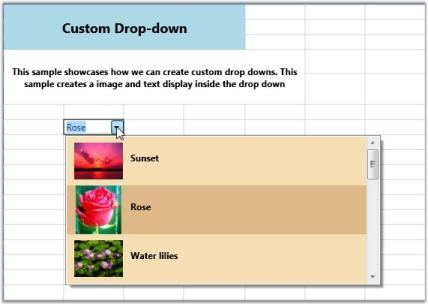Custom Drop-down

NOTE

For complete code, please refer to the following browser sample. …\My Documents\Syncfusion\EssentialStudio<Version Number>\WPF\Grid.WPF\Samples\3.5\WindowsSamples\Cell Types\Custom Drop Down Demo

### Data Template Cells

This cell builds a custom data template that can be used to set enriched styles for associated cells. The DataTemplateCellModel creates the cell type with a Content Control (a WPF control) by calling the renderer.

NOTE

To create a cell type that hosts a WPF control, you should derive it from GridVirtualizingCellRenderer. The most important method to override is the OnInitializeContent method. It will be called for every UI element created for cells displaying this renderer. You can get access to the cell style from this method.

The DataTemplateCellRenderer is derived from GridVirtualizingCellRenderer and overrides OnInitializeContent and sets the Content Control template to Style.CellItemTemplate value.

#### CellModel Class

``````public class DataTemplateCellModel : GridCellModel<DataTemplateCellRenderer>
{
}``````

#### CellRenderer Class

``````public class DataTemplateCellRenderer : GridVirtualizingCellRenderer<ContentControl>
{

public DataTemplateCellRenderer()
{
IsFocusable = true;
AllowRecycle = true;
}

public override void OnInitializeContent(ContentControl uiElement, GridRenderStyleInfo style)
{
base.OnInitializeContent(uiElement, style);
bool found = false;

if (style.CellItemTemplateKey != null)
{
DataTemplate dt = (DataTemplate)style.GridControl.TryFindResource(style.CellItemTemplateKey);
found = dt != null;

if (found)
uiElement.ContentTemplate = dt;
}

if (!found)
uiElement.ContentTemplate = style.CellItemTemplate;

uiElement.Content = style.CellValue;
}

public override void CreateRendererElement(ContentControl uiElement, GridRenderStyleInfo style)
{

bool found = false;

if (style.CellItemTemplateKey != null)
{
DataTemplate dt = (DataTemplate)style.GridControl.TryFindResource(style.CellItemTemplateKey);
found = dt != null;
if (found)
uiElement.ContentTemplate = dt;
}

if (!found)
uiElement.ContentTemplate = style.CellItemTemplate;

uiElement.Content = style.CellValue;
base.CreateRendererElement(uiElement, style);
}

protected override string GetControlTextFromEditorCore(ContentControl uiElement)
{
return uiElement.Content.ToString();
}
}``````

#### Data Template Definition

``````<DataTemplate x:Key="editableEmployee">
<StackPanel Margin="8,0"  Orientation="Horizontal">
<TextBlock FontWeight="Bold" syncfusion:VisualContainer.WantsMouseInput="False" Text="{Binding Path=Name}" Width="70" />
<TextBox Text="{Binding Path=Title}" BorderThickness="0"  Padding="0" Margin="0" Width="130" x:Name="tb"/>
</StackPanel>
</DataTemplate>``````

#### Setting up the Data Template Cell and assigning the Cell Template

``````grid.Model.CellModels.Add("DataTemplate", new DataTemplateCellModel());
grid.Model.QueryCellInfo += new Syncfusion.Windows.Controls.Grid.GridQueryCellInfoEventHandler(Model_QueryCellInfo);

void Model_QueryCellInfo(object sender, Syncfusion.Windows.Controls.Grid.GridQueryCellInfoEventArgs e)
{

if (e.Cell.RowIndex > 1 && e.Cell.ColumnIndex == 2)
{
e.Style.CellType = "DataTemplate";
e.Style.CellItemTemplateKey = "editableEmployee";
e.Style.CellValue = employeesSource.Employees[e.Cell.RowIndex % employeesSource.Employees.Count];
e.Style.Background = Brushes.Linen;
}
}``````

#### Output

The following output is generated using the code above.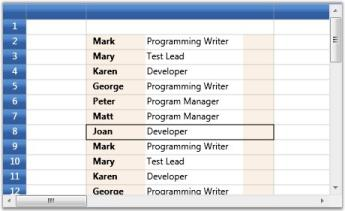Data Template with Cell Template Assigned

NOTE

For complete code, please refer to the following browser sample. …\My Documents\Syncfusion\EssentialStudio<Version Number>\WPF\Grid.WPF\Samples\3.5\WindowsSamples\Cell Types\Data Template Cell Demo

### Rich Text Box Cells

The Rich Text control will allow you to display and edit rich text in grid cells. The control will allow you to modify the rich text through in-place editing.

#### Example

It can be built by hosting the Rich Text Box control in grid cells. To host this control, the cell renderer must be derived from GridVirtualizingCellRenderer, whose OnInitializeContent should be overridden to provide the content (as Flow Document) for the rich text box.

#### CellModel class

``````public class RichTextBoxCellModel : GridCellModel<RichTextBoxCellRenderer>
{
}``````

#### CellRenderer class

``````public class RichTextBoxCellRenderer : GridVirtualizingCellRenderer<RichTextBox>
{

public RichTextBoxCellRenderer()
{
IsControlTextShown = false;
IsFocusable = true;
}

public override void OnInitializeContent(RichTextBox textBox, GridRenderStyleInfo style)
{
FlowDocument document = GetControlValue(style) as FlowDocument;

if (document == null)
textBox.Document = new FlowDocument();

if (document.Parent != null)
{
var parentTextBox = document.Parent as RichTextBox;

parentTextBox.Document = new FlowDocument();
}
textBox.Document = document;
VirtualizingCellsControl.SetWantsMouseInput(textBox, true);
}

protected override void OnUnwireUIElement(RichTextBox uiElement)
{
uiElement.Document = new FlowDocument();
base.OnUnwireUIElement(uiElement);
}

protected override object GetControlValueFromEditorCore(RichTextBox uiElement)
{
return uiElement.Document;
}

protected override void OnGridPreviewTextInput(TextCompositionEventArgs e)
{
CurrentCell.ScrollInView();
CurrentCell.BeginEdit(true);
}

protected override bool ShouldGridTryToHandlePreviewKeyDown(KeyEventArgs e)
{

if (CurrentCellUIElement.IsFocused && e.Key != Key.Escape)
return false;
return true;
}
}``````

#### Setting up Rich Text Box Cell

``````grid.Model.CellModels.Add("RichText", new RichTextBoxCellModel());
{

// Create a FlowDocument to contain content for the RichTextBox.
FlowDocument myFlowDoc = new FlowDocument();

// Add paragraphs to the FlowDocument.
grid.Model[2, 2].CellType = "RichText";
grid.Model[2, 2].CellValue = myFlowDoc;
}
{
Paragraph myParagraph = new Paragraph();

// Add some Bold text to the paragraph
myParagraph.Inlines.Add(new Bold(new Run("Some bold text in the paragraph.")));

// Add some plain text to the paragraph
myParagraph.Inlines.Add(new Run(" Some text that is not bold."));

// Create a List and populate with three list items.
List myList = new List();

// First create paragraphs to go into the list item.
Paragraph paragraphListItem1 = new Paragraph(new Run("ListItem 1"));
Paragraph paragraphListItem2 = new Paragraph(new Run("ListItem 2"));
Paragraph paragraphListItem3 = new Paragraph(new Run("ListItem 3"));

// Add ListItems with paragraphs in them.

// Create a FlowDocument with the paragraph and list.
FlowDocument myFlowDocument = new FlowDocument();

grid.Model[10, 2].CellType = "RichText";
grid.Model[10, 2].CellValue = myFlowDocument;
}``````

#### Output

The following output is generated using the code above.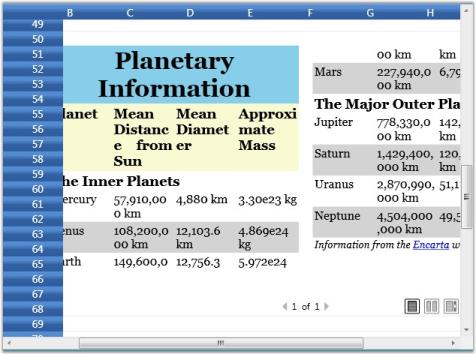Rich Text Box Cell

### Chart Cells

Grid provides inherent support to host chart controls. This is achieved by using Data Template cells.

#### Example

1. Define the Data Template that creates a chart. The template below illustrates the creation of a chart control with a single series and defines its attributes.
``````<local:MyDataCollection x:Key="SeriesData1"/>
<DataTemplate x:Key="DataChart">
<!--Hosting Chart control in second Row of the Grid-->
<syncfusion:Chart   x:Name="Chart1" Grid.Row="1" Margin="15">
<!--Chart Legend declaration-->
<syncfusion:Chart.Legends>
<syncfusion:ChartLegend />
</syncfusion:Chart.Legends>
<!--Chart area to present chart segments-->
<!--Primary Axis(X)-->
<syncfusion:ChartArea.PrimaryAxis>
<!--X axis declaration with required property settings-->
</syncfusion:ChartAxis>
</syncfusion:ChartArea.PrimaryAxis>
<!--Secondary Axis(Y)-->
<syncfusion:ChartArea.SecondaryAxis>
<!--Y axis declaration with required property settings-->
</syncfusion:ChartAxis>
</syncfusion:ChartArea.SecondaryAxis>
<!--Chart 1st series declaration-->
<syncfusion:ChartSeries Name="series1" Label="Profit in \$"  Type="Spline" StrokeThickness=" 3" Interior="Green"  DataSource="{StaticResource SeriesData1}"
BindingPathX="Year" BindingPathsY="Y1" IsIndexed="False">
</syncfusion:ChartSeries>
</syncfusion:ChartArea>
</syncfusion:Chart>
</DataTemplate >``````

Here is the data source definition that is used to define the chart series.

``````public class MyData
{
public int Year { get; set; }
public double Y1 { get; set; }
public double Y2 { get; set; }
public double Y3 { get; set; }
public double Y4 { get; set; }
}

public class MyDataCollection : ObservableCollection<MyData>
{

public MyDataCollection()
{
Random rand = new Random(DateTime.Now.Millisecond);

for (int i = 0; i < 5; i++)
{
{
Y1 = rand.Next(700, 1200),
});
}
}
}``````
1. Bind the above template to the grid cell to form a chart cell.
``````var cell = grid.Model[2, 2];
cell.CellType = "DataTemplate";
cell.CellItemTemplateKey = "DataChart";
grid.Model.RowHeights = 400d;``````

#### Output

The following output is generated using the code above.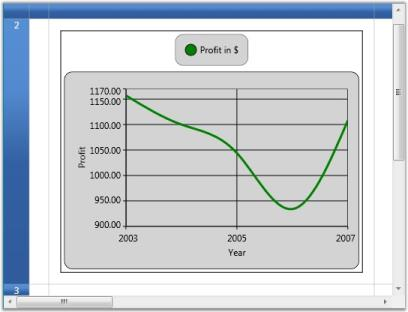Chart Cell

## Inserting Images into Grid Cells

Grid provides inherent support to add images into grid cells. There are two possible ways to achieve this.

• Style.Image property—Used to insert an image alongside the text in the grid cells.
• Style.ImageList property—It serves the same purpose as the Image property, points to a collection of images and lets you share the same ImageSource for a group of cells. Once you have selected the images by using the ImageList property, you must set the ImageIndex property for individual cells, to indicate the specific image (from the ImageList) to be inserted into the cell.

NOTE

If you use both Image and ImageList properties, then the most recent property applied will be considered.

The following code example illustrates how to use these properties.

``````// Using Vector Images.
ResourceDictionary dictionary = new ResourceDictionary();
dictionary.Source = new Uri(vectorImgSrcUri, UriKind.RelativeOrAbsolute);
ObservableCollection<Image> imgList = new ObservableCollection<Image>();

foreach (string key in dictionary.Keys)
{
Image img = new Image();
img.Source = (DrawingImage)dictionary["Technology"];
}

// Setting ImageList.
grid.Model.TableStyle.ImageList = imgList;

// Image property setting.
grid.Model[0, 2].Text = "Technology";
Image img = new Image();
img.Source = new BitmapImage(new Uri("Technology.png", UriKind.Absolute));
grid.Model[0, 2].Image = img;

// Setting ImageIndex.